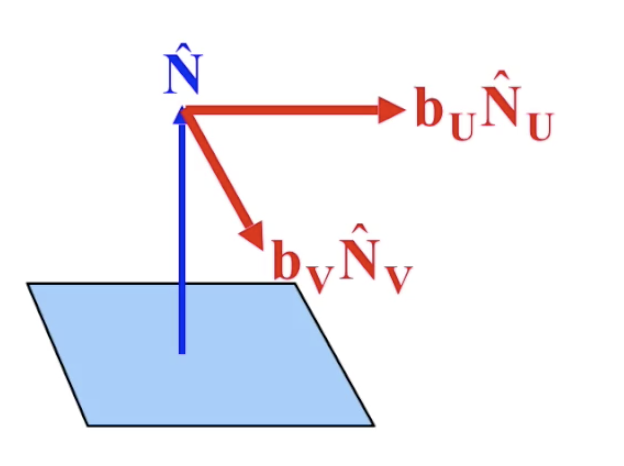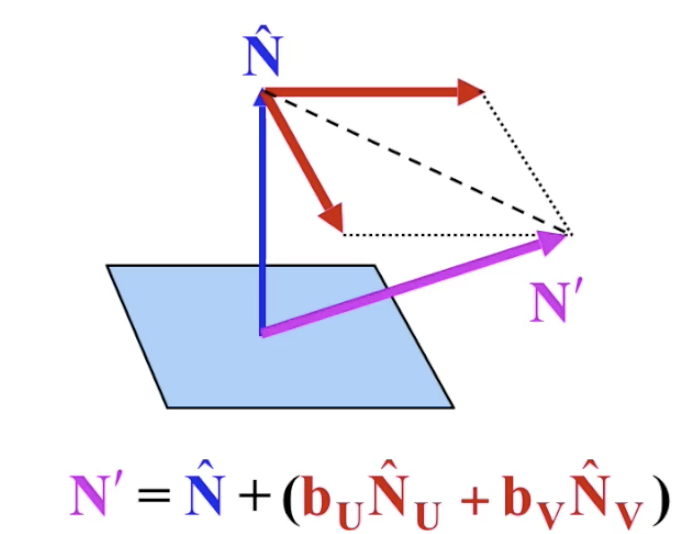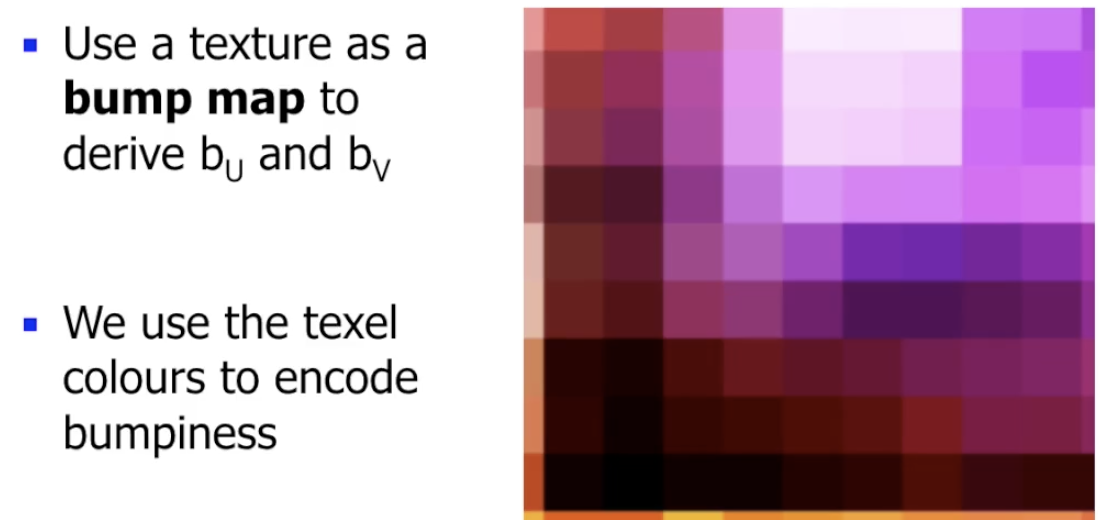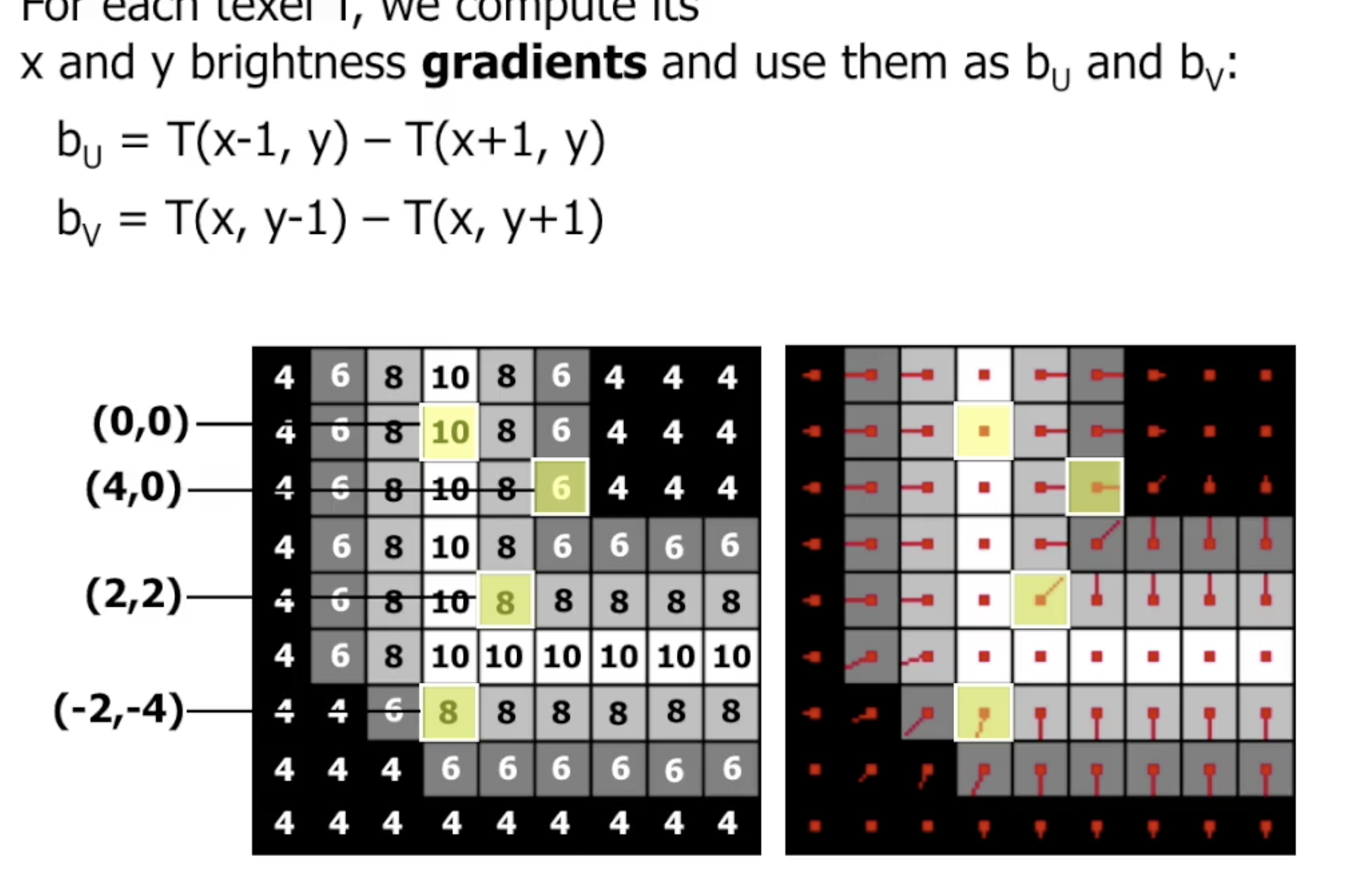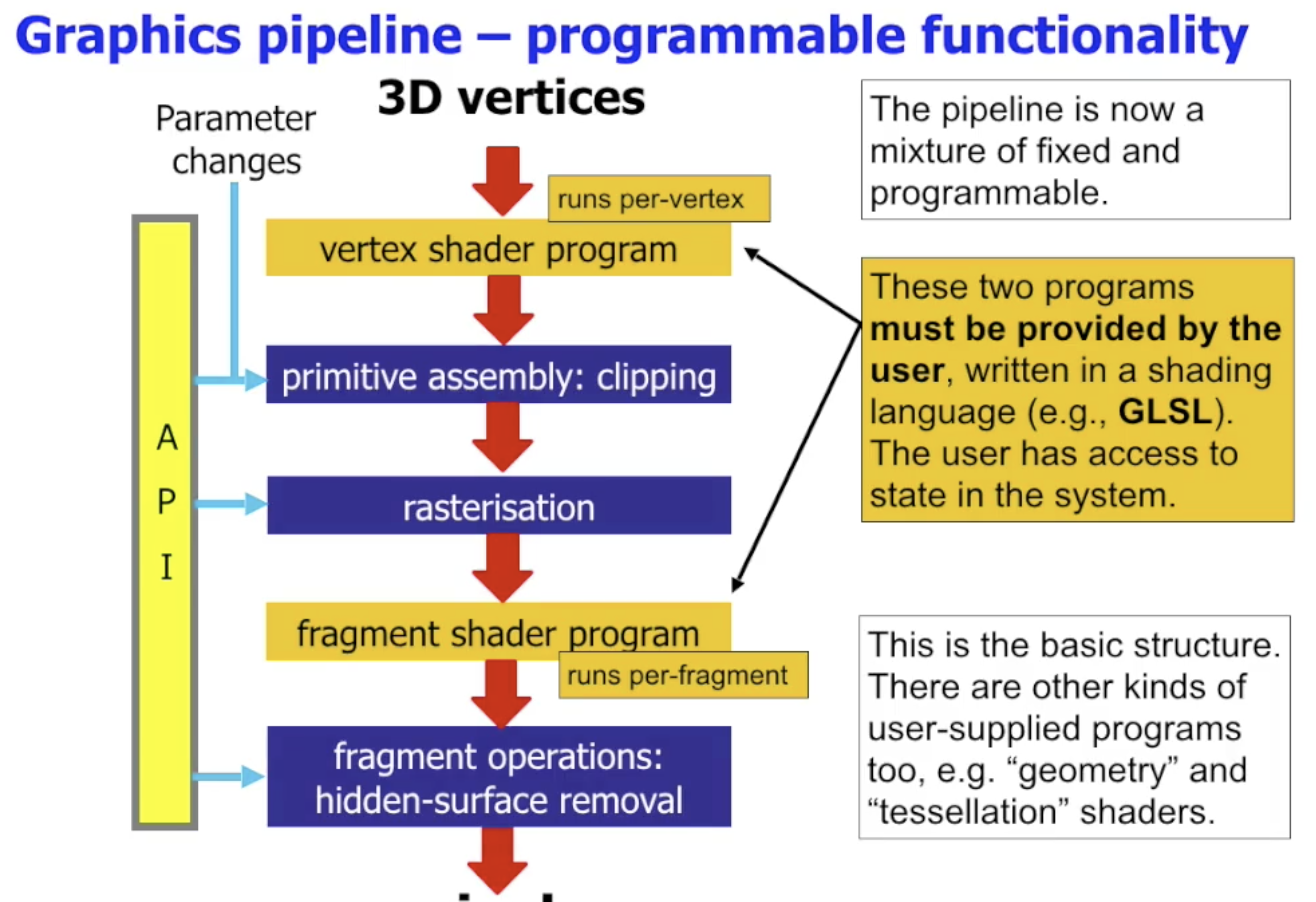# 计算机图形学导论：渲染

## Rendering

Posted by R1NG on March 15, 2022 Viewed Times

# 渲染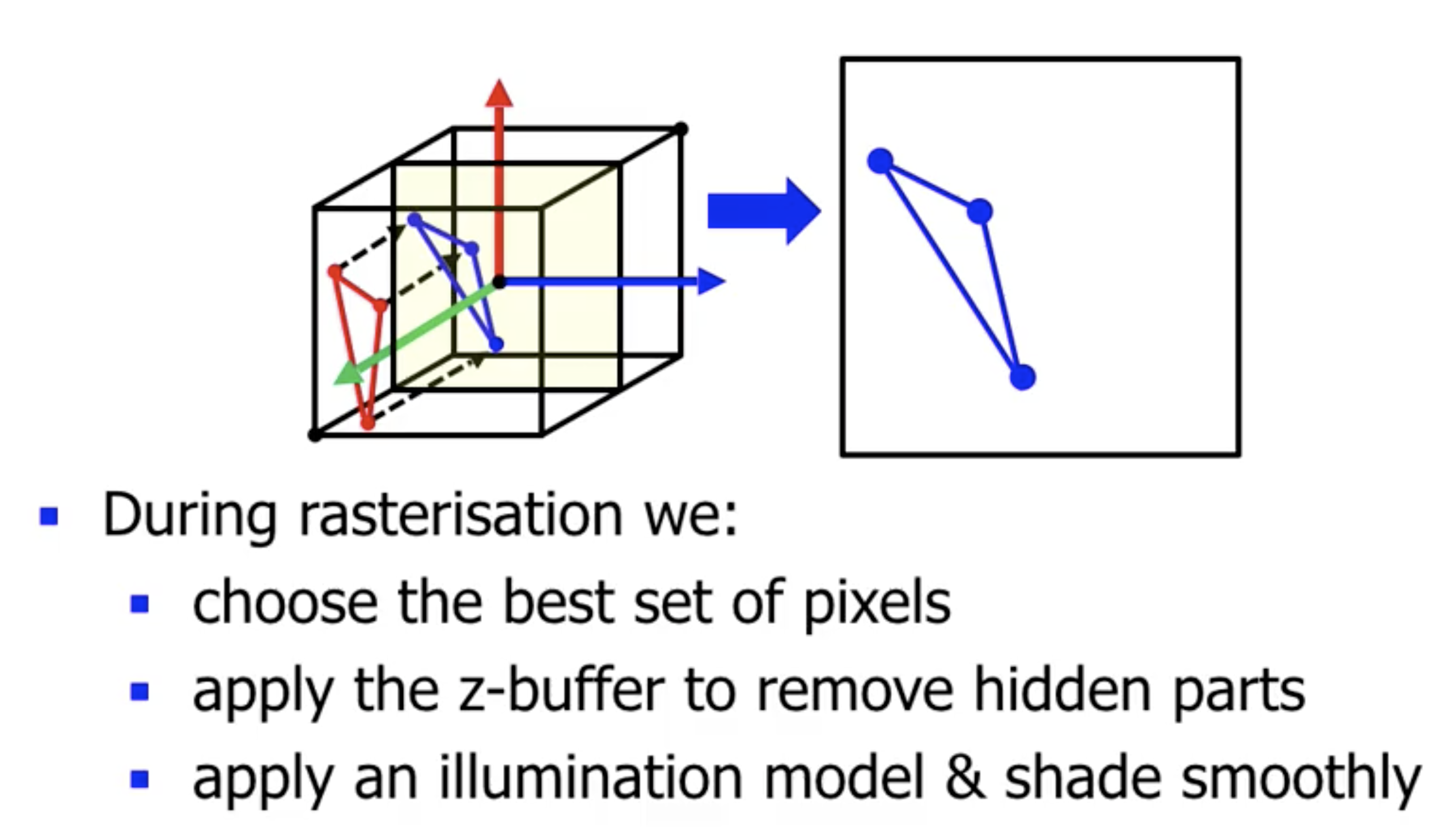ThreeJS 中, 光照模型和上色方式的选定 被封装在声明三维物体时的 材质选择 中: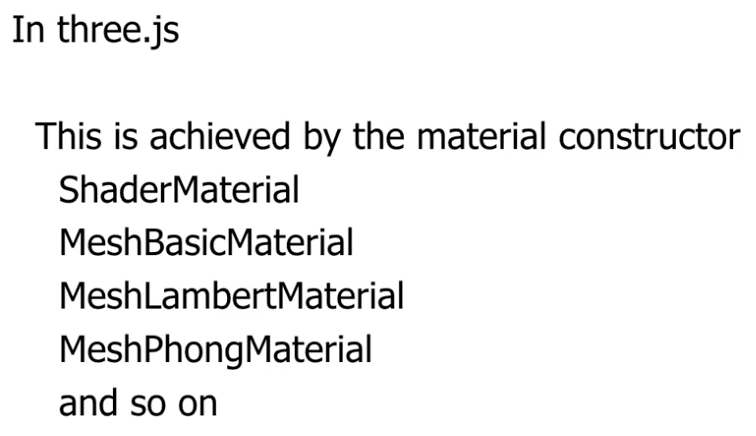## 光照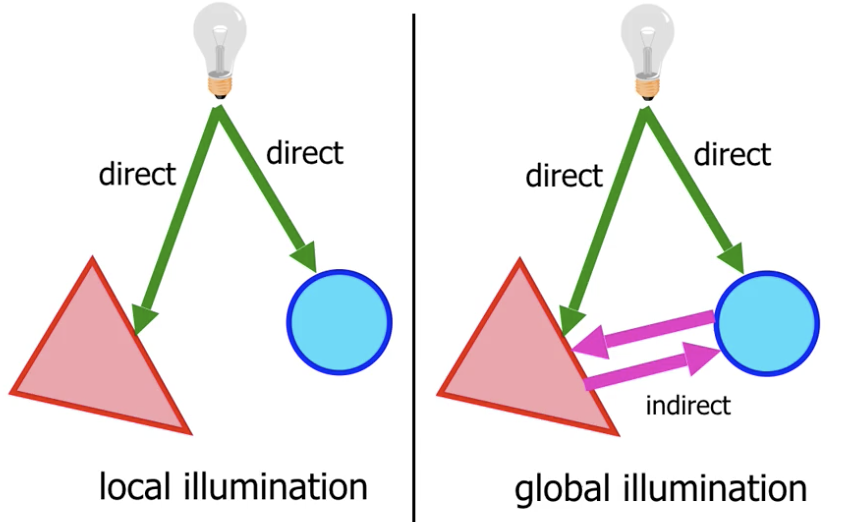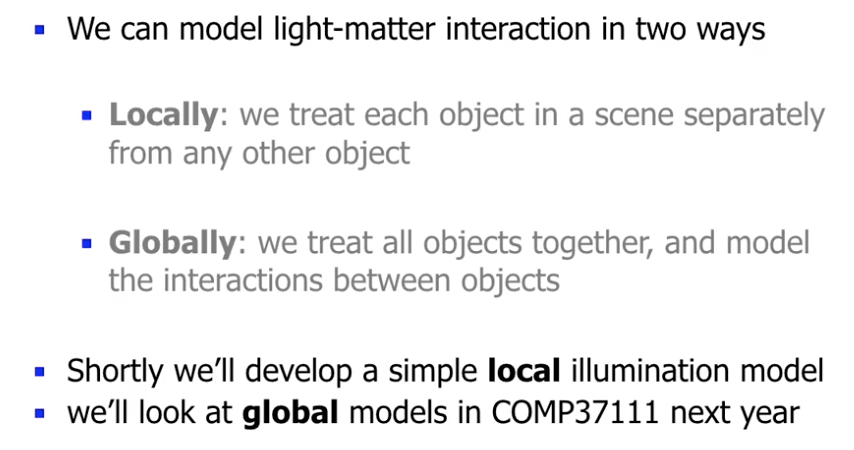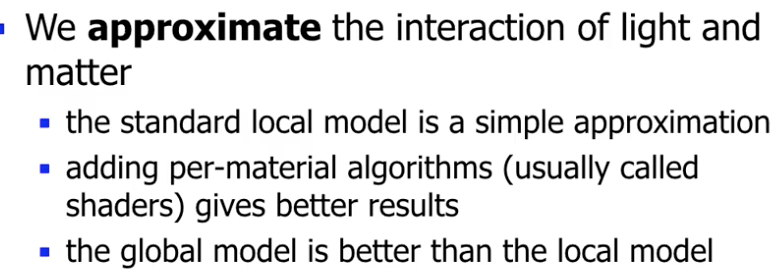## 反射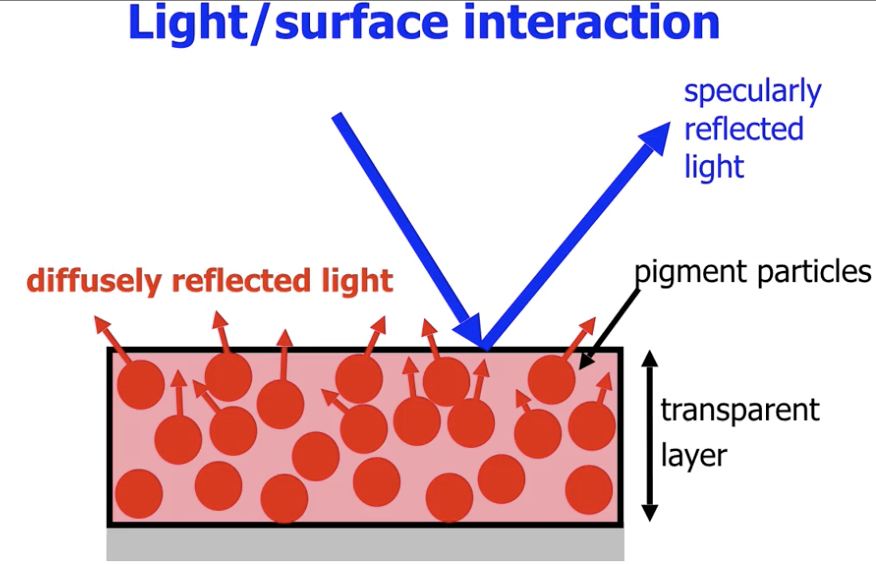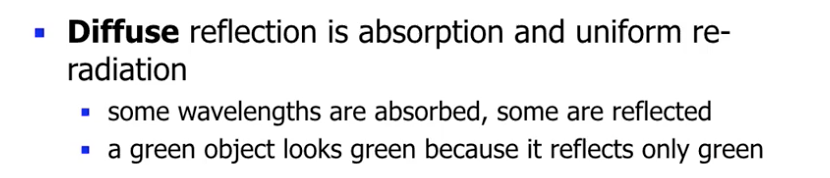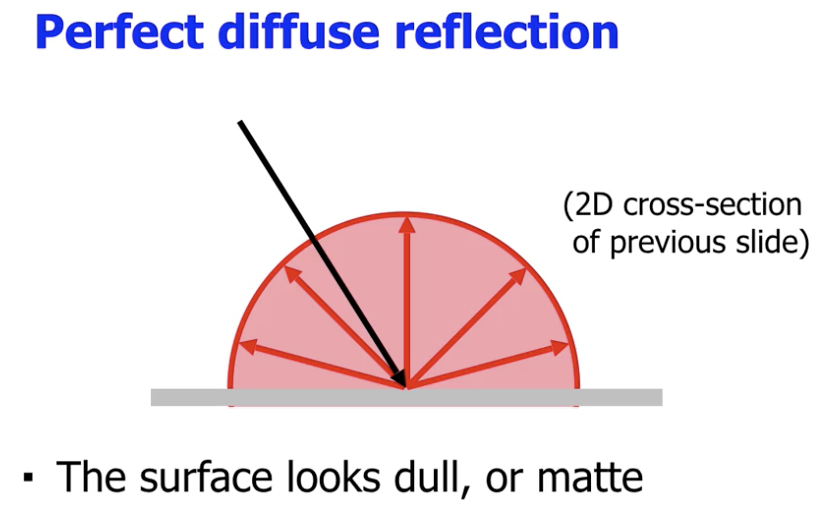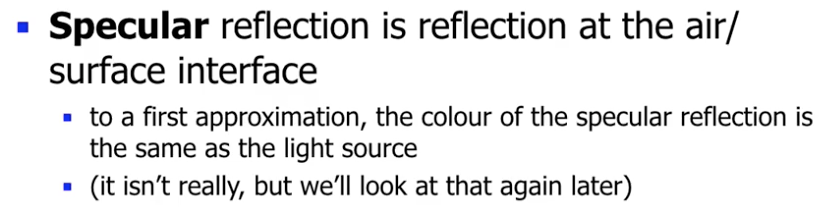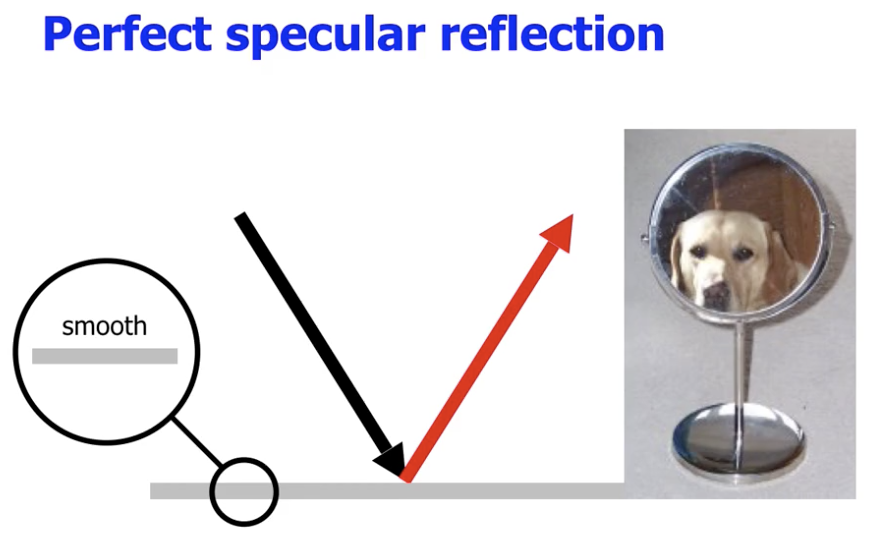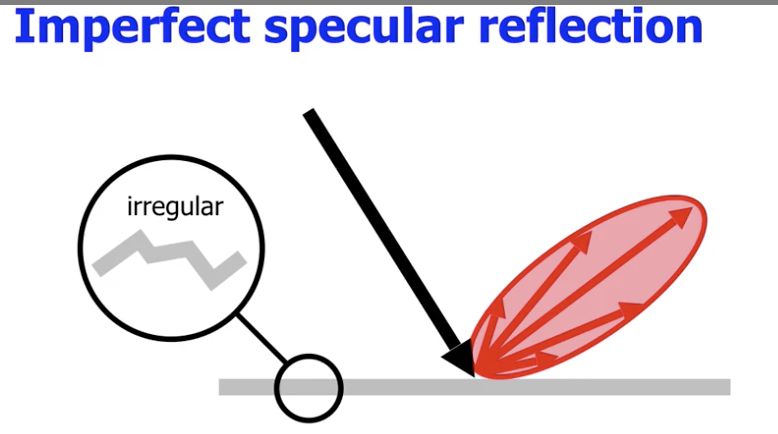### 建模漫反射 (Diffuse Reflection)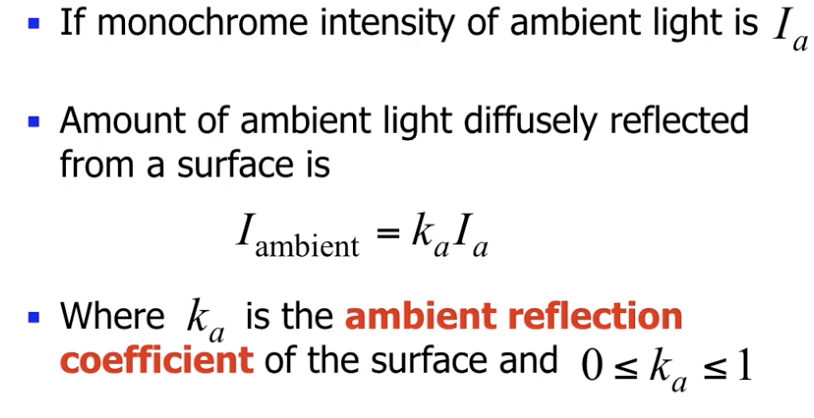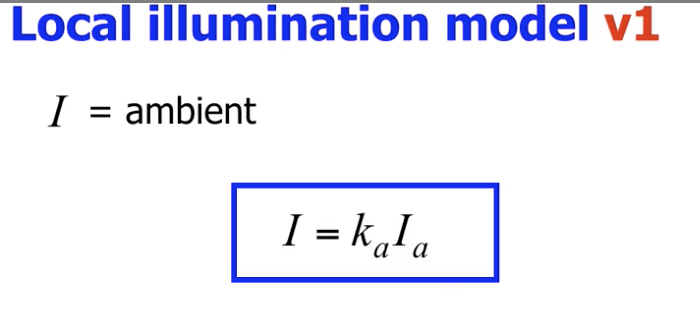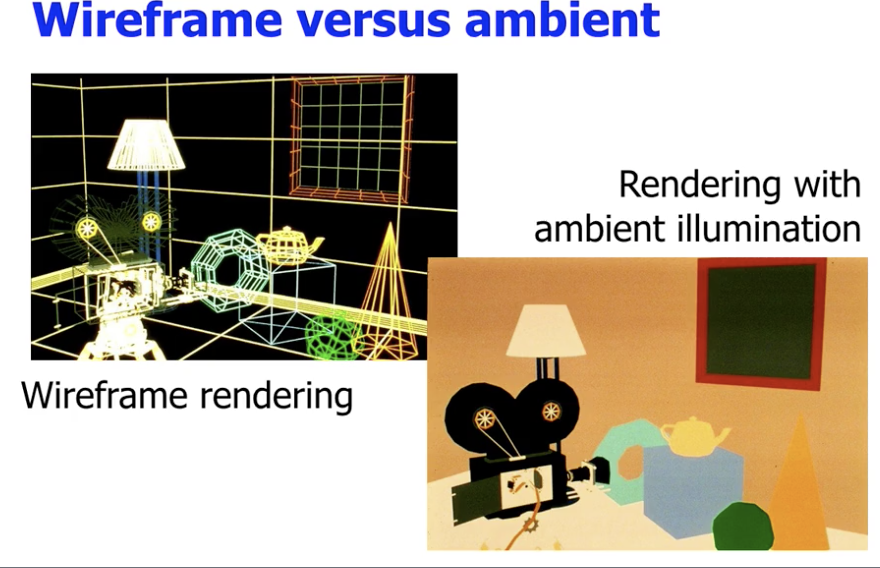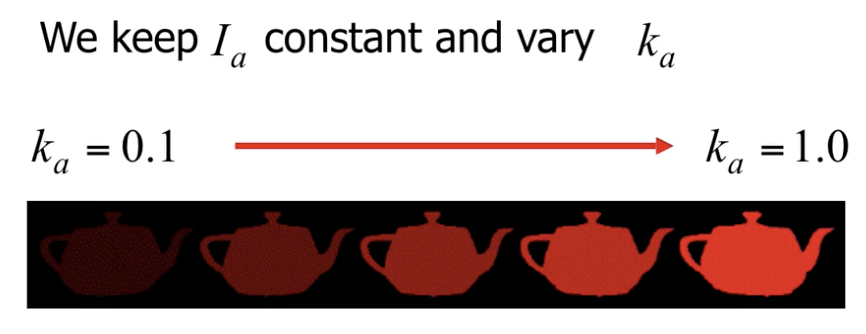1. 光照强度随距离光源远近的衰减
2. 光线入射角随距离光源远近的变化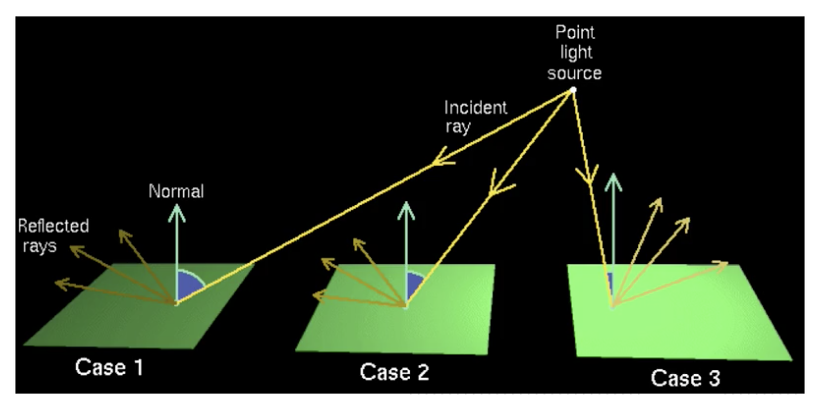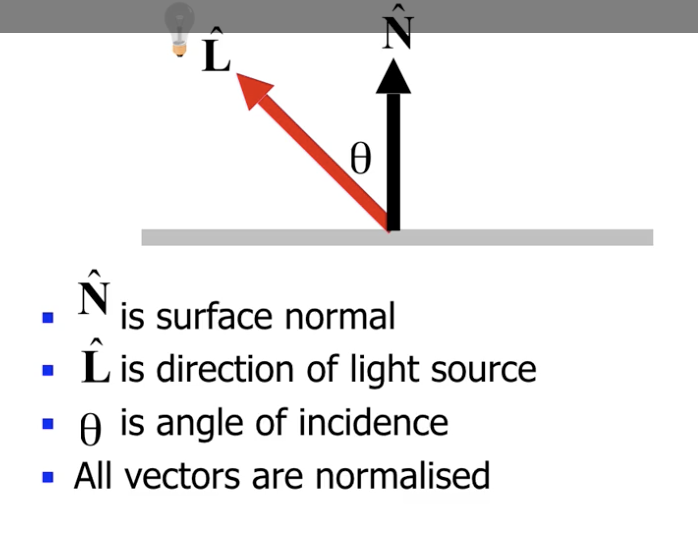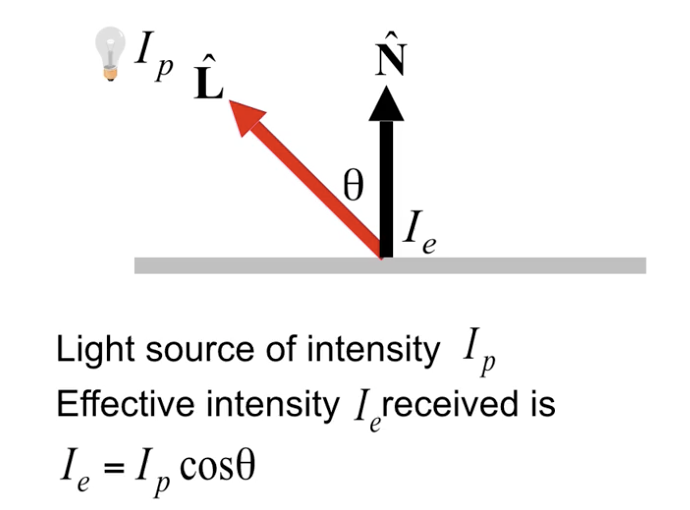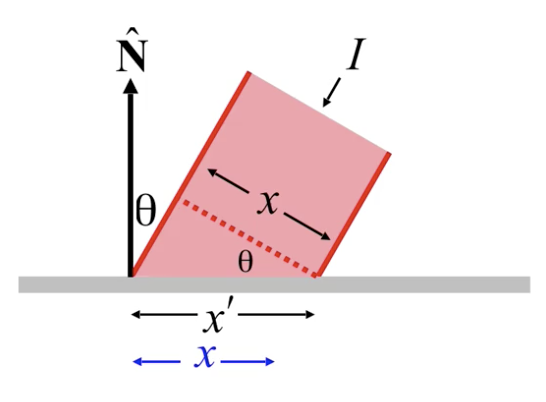$I_{\text{diffuse}} = I_p \cdot k_d \cdot(N \cdot L).$

(因为 $N, L$ 都是单位向量, 所以 $N\cdot L = \vert N\vert \cdot \vert L \vert \cdot \cos(\theta) = \cos(\theta)$.)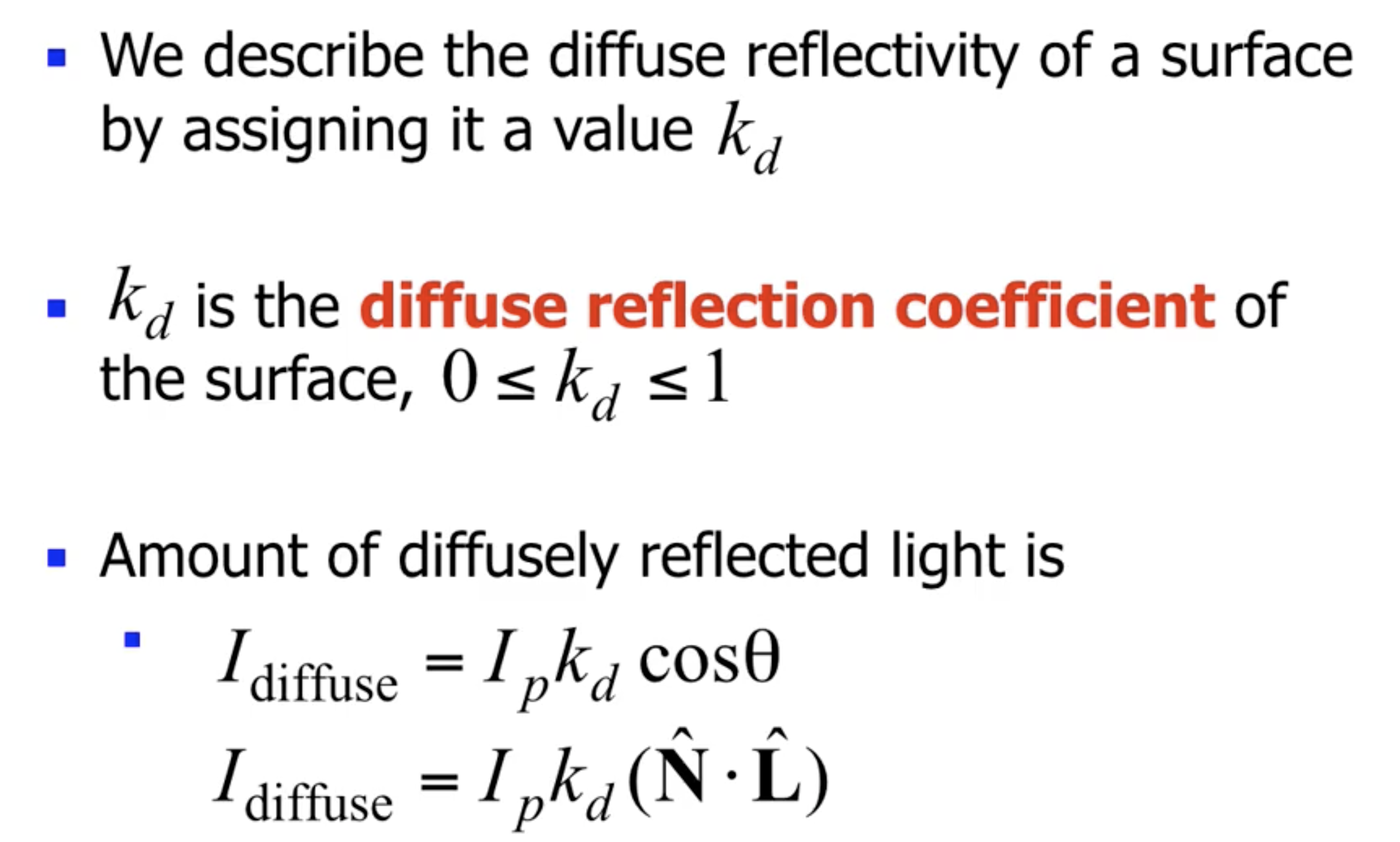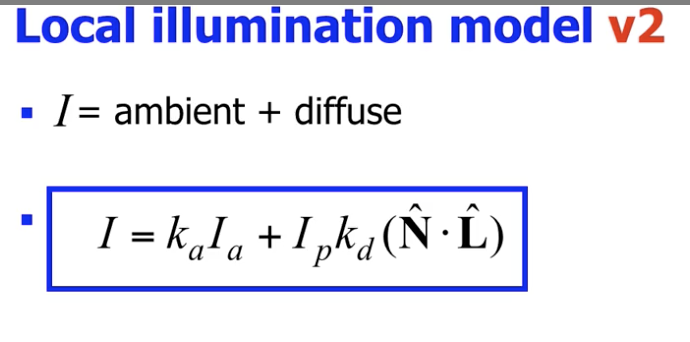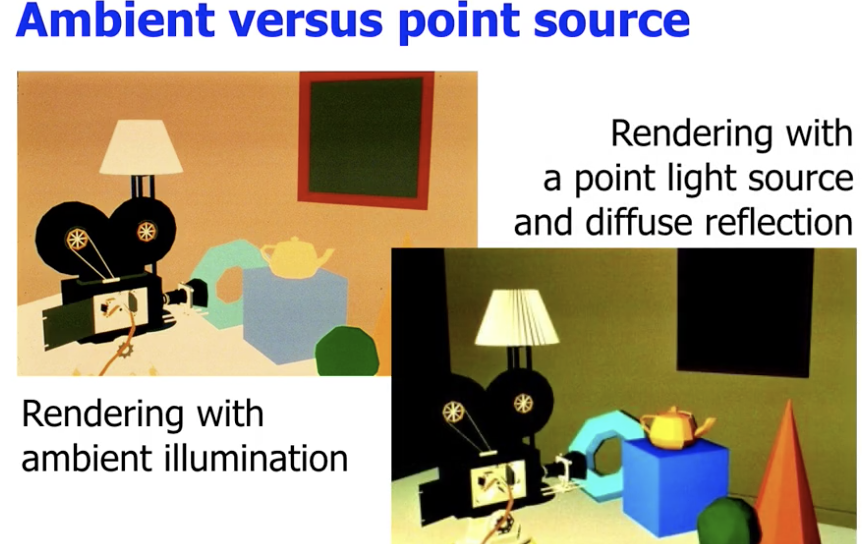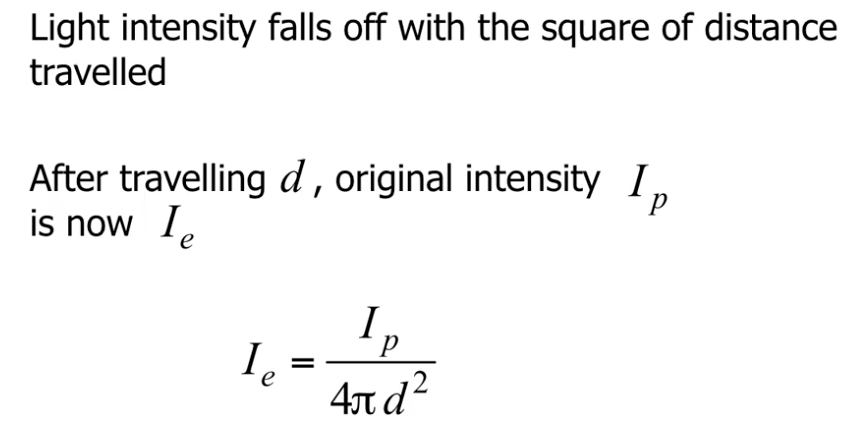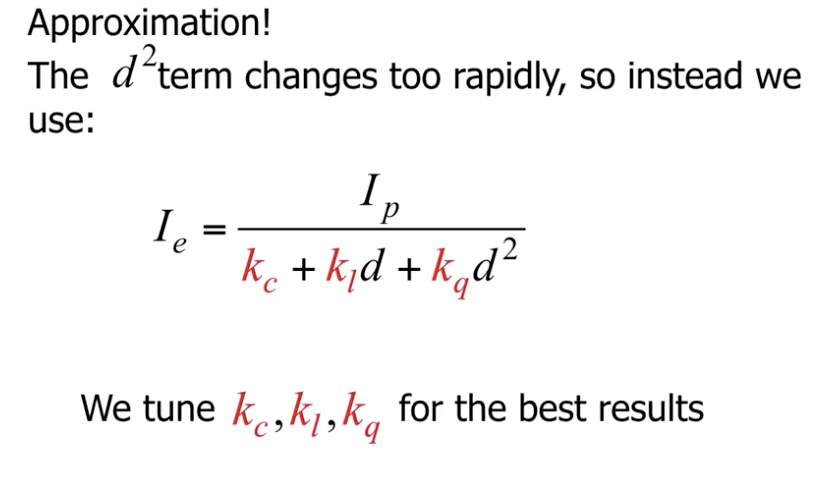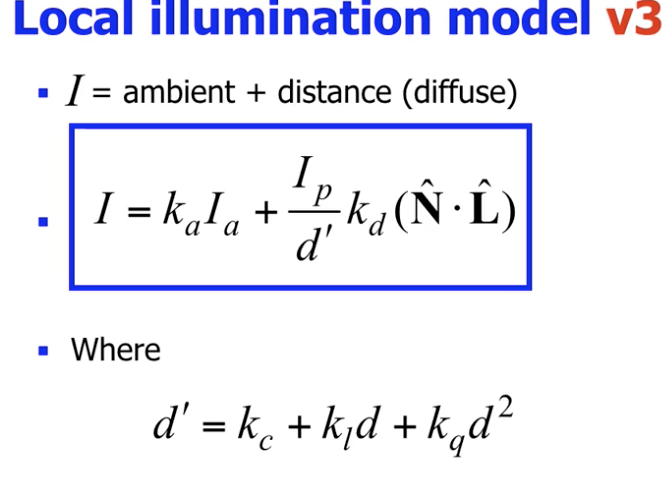### 建模镜面反射 (Specular Reflection)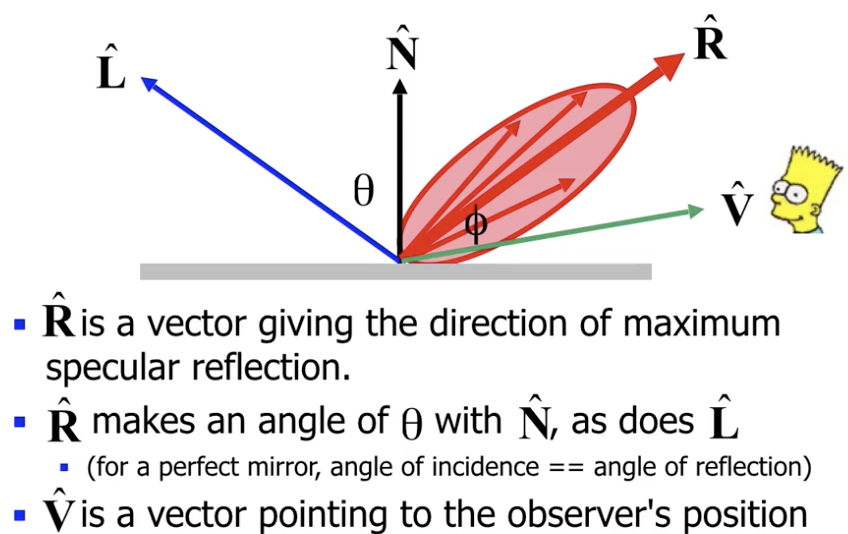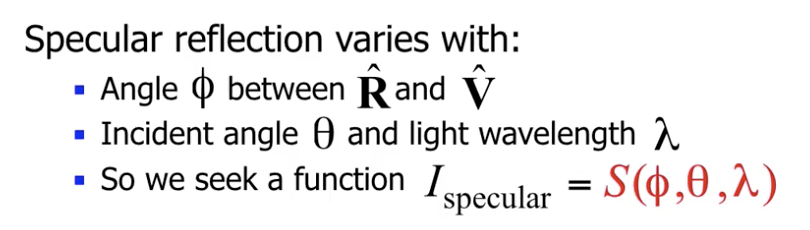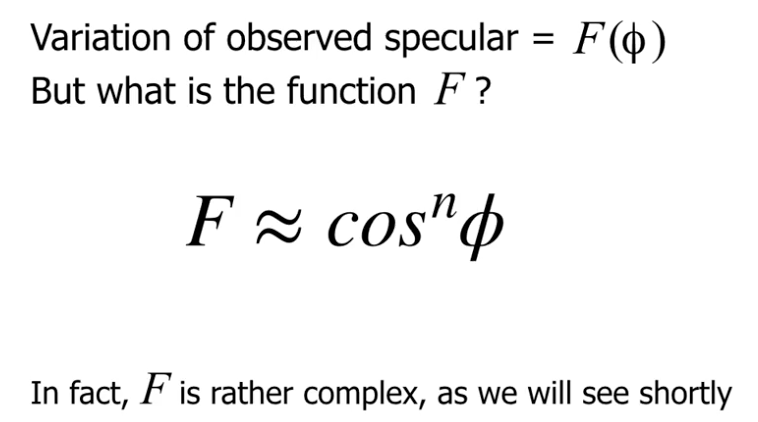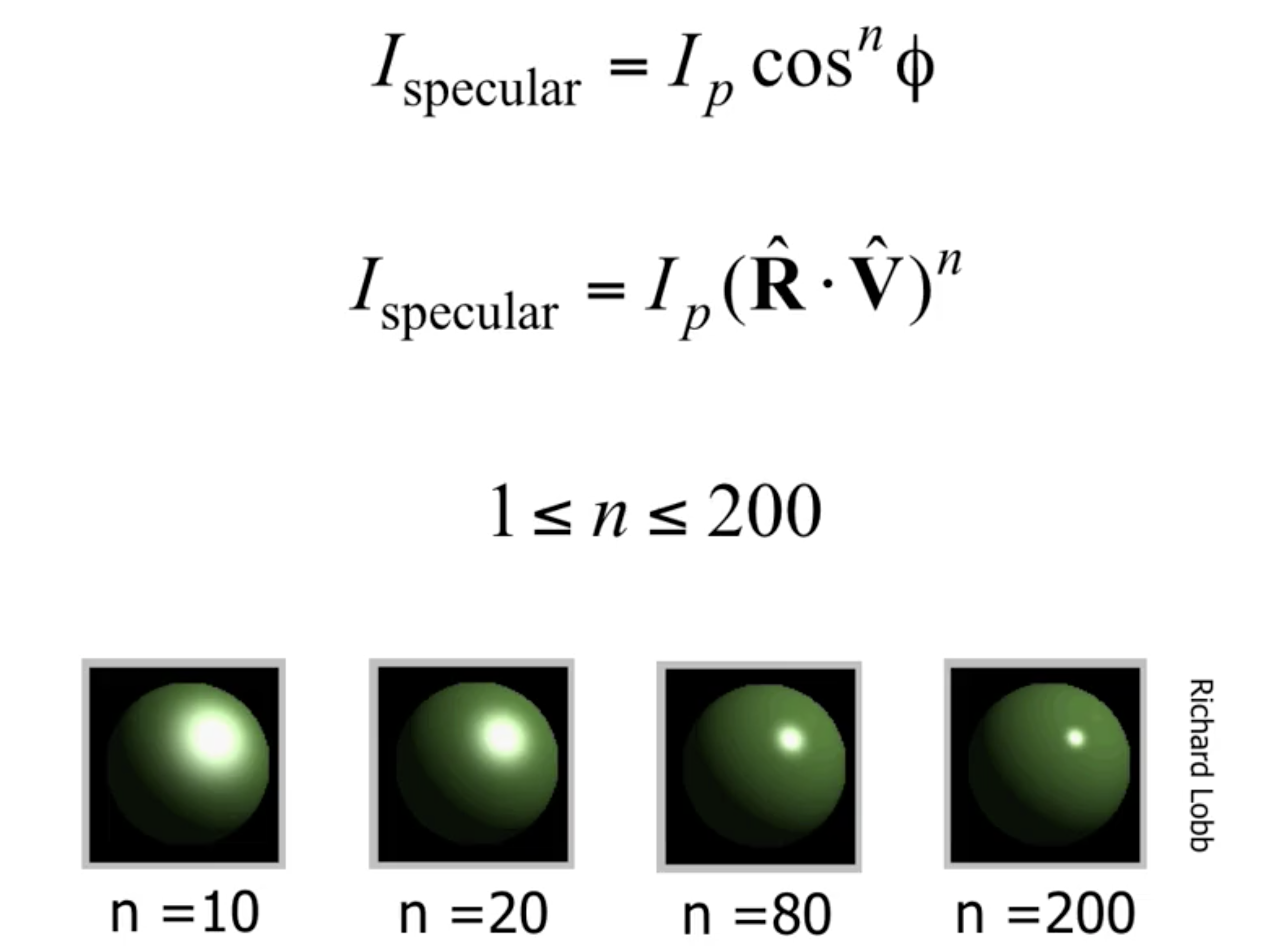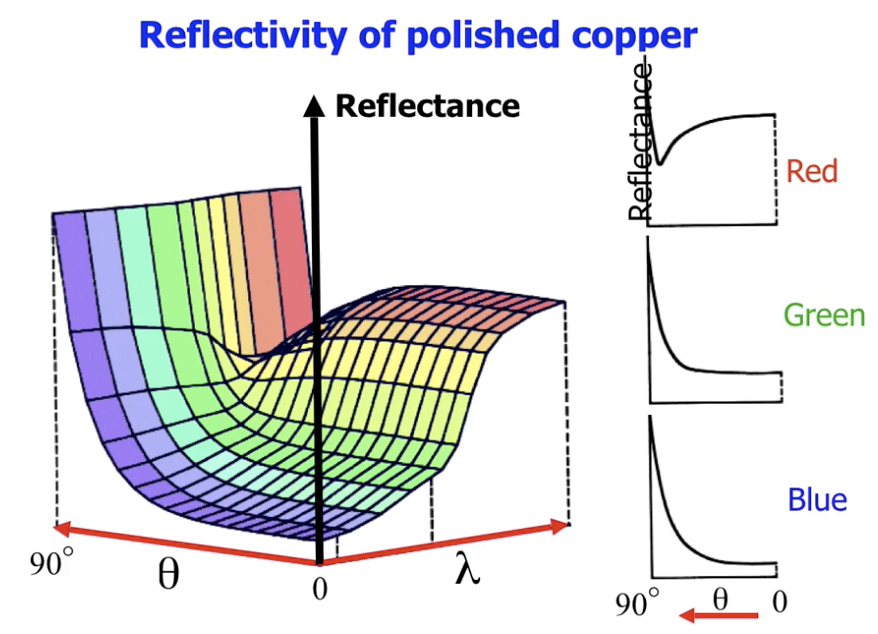$\sin(\theta) = \frac{\sin(\phi)}{\mu}.$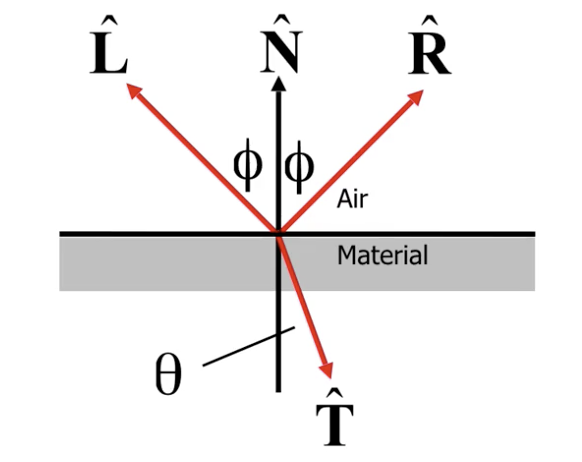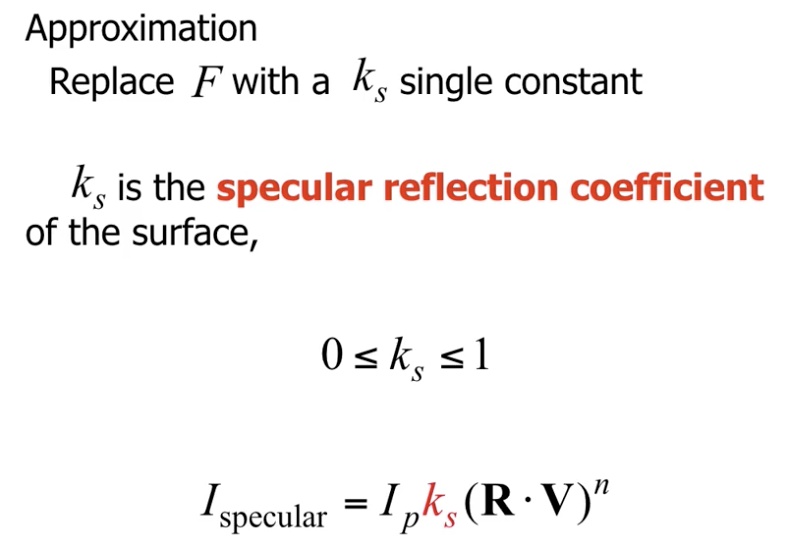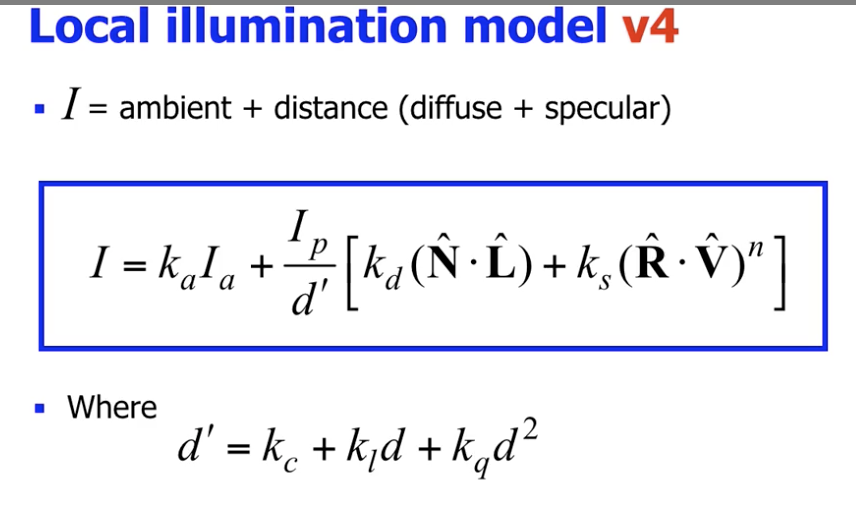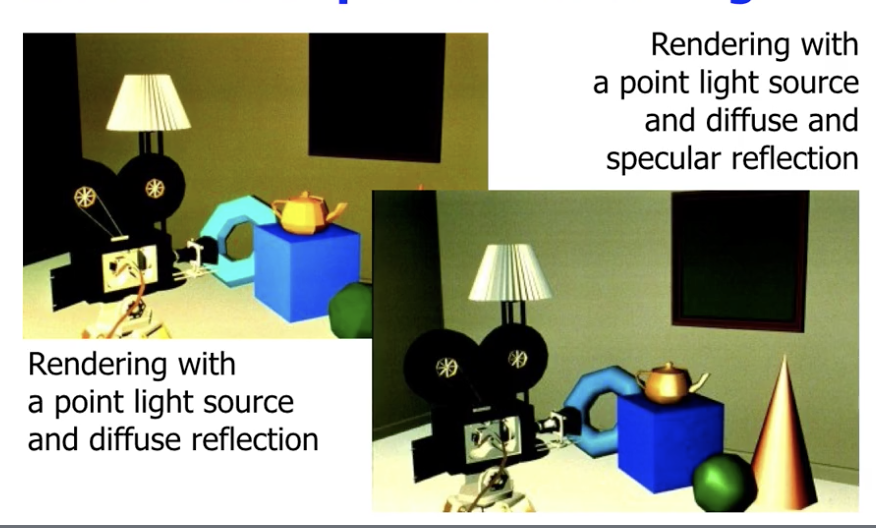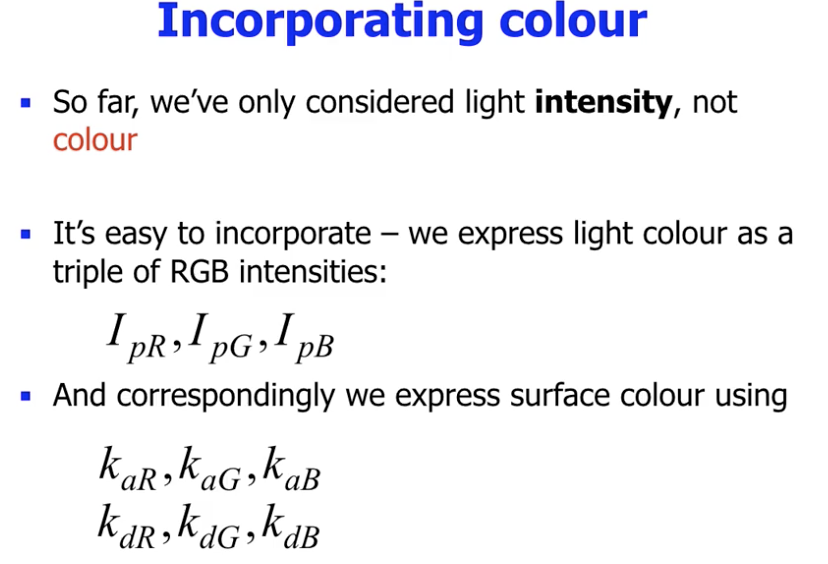1. 环境光漫反射系数 $k_a$ 和局部光漫反射系数 $k_d$ 因颜色通道而异!
2. 镜面反射系数 $k_s$ 不受颜色通道影响!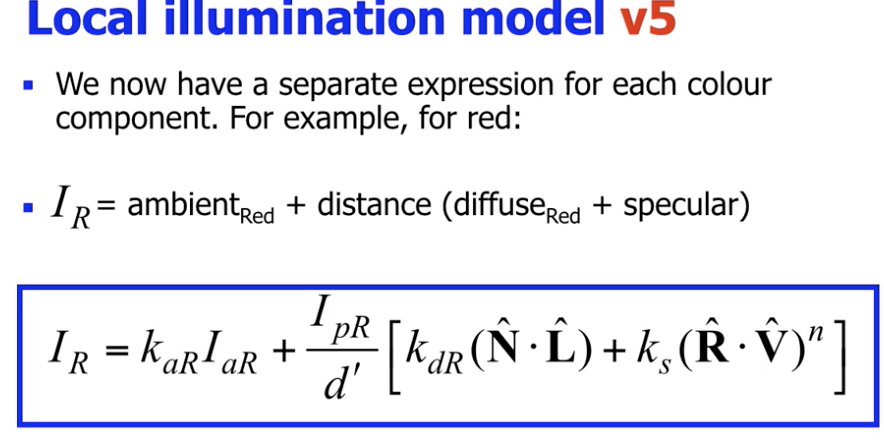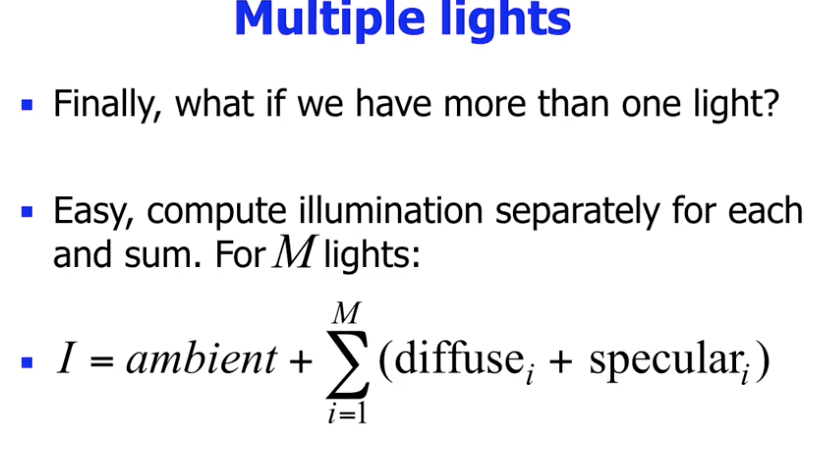## 着色

### Flat Shading

Flat Shading 又称 Constant Shading, 在着色时, 使用 该面的法线 结合光照模型计算颜色, 然后将这个颜色 应用在整个面上.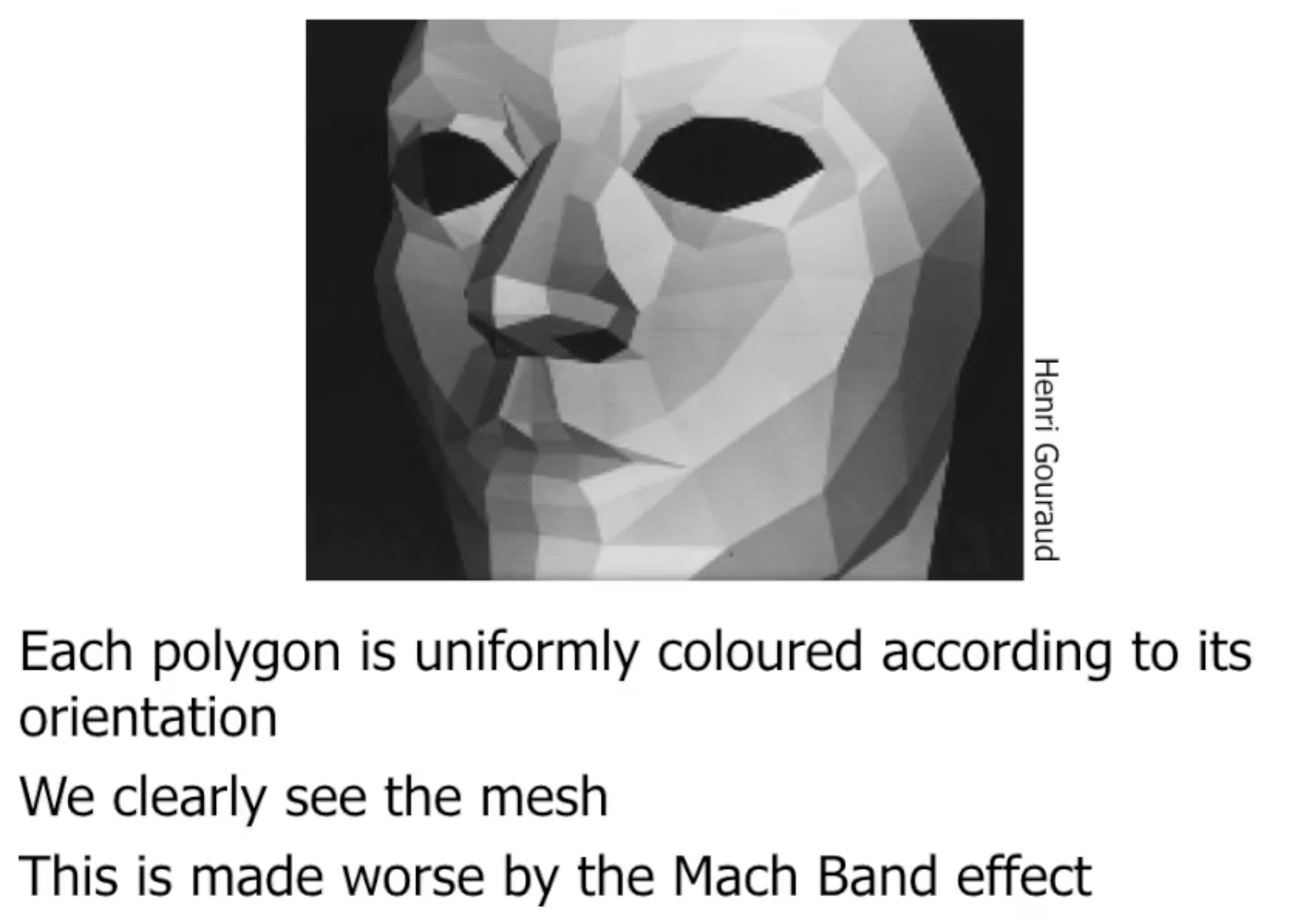### Gouraud Shading

Gouraud Shading 使用 插值 尝试 模糊化面法线不同的面之间颜色的区别, 减少割裂感.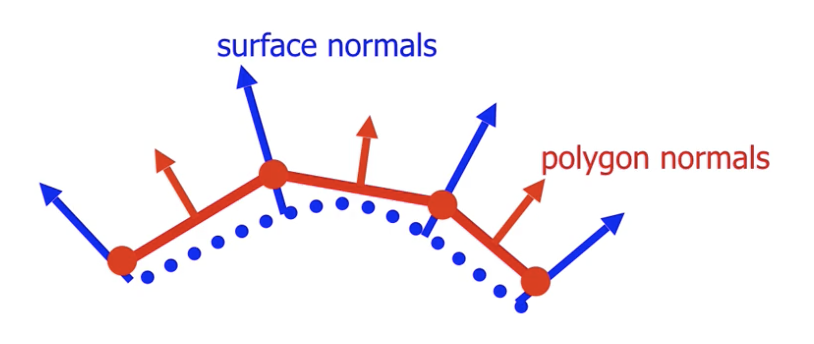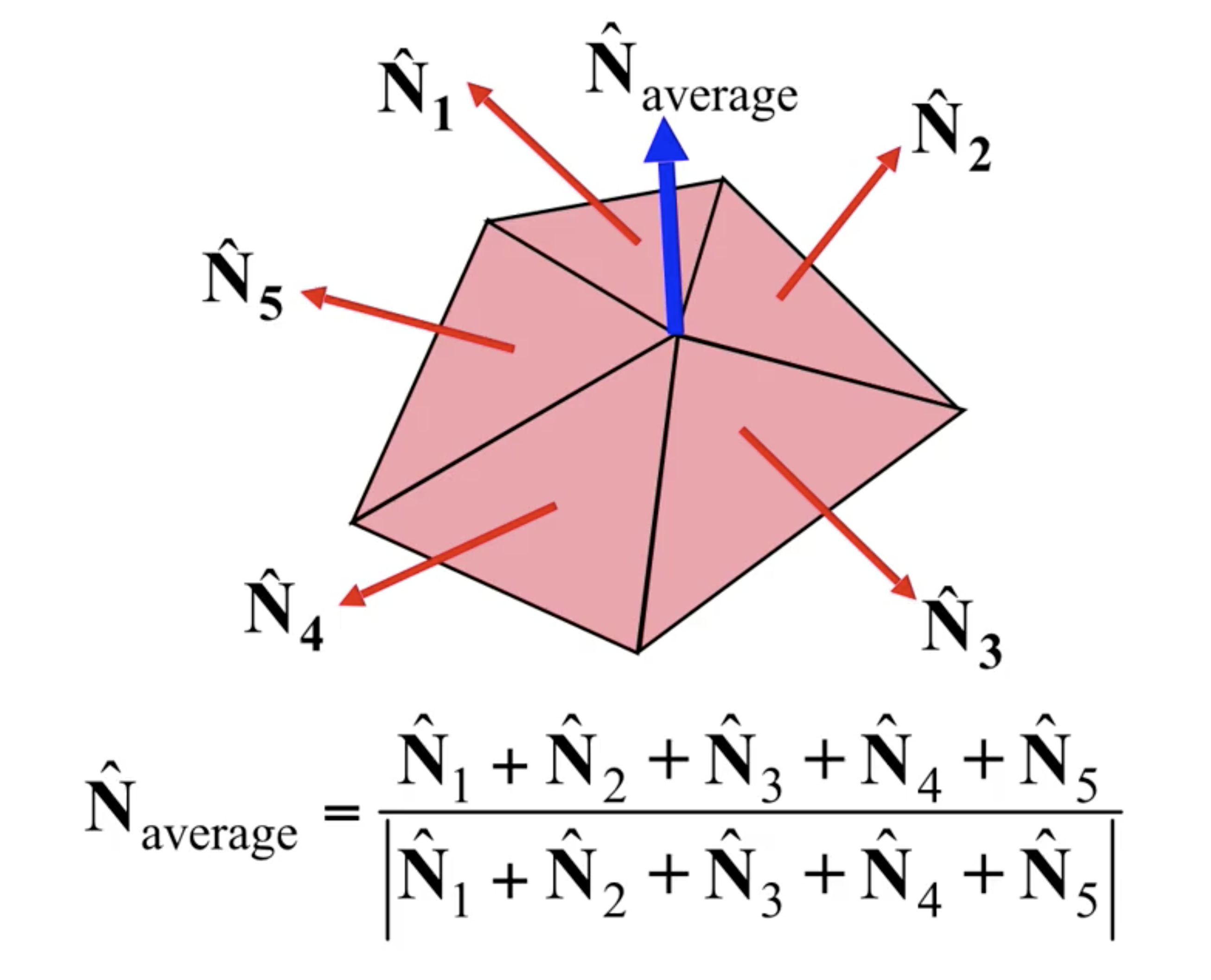1. 假设平滑方向是自左向右, 首先分别关于左侧边 $AC$, 基于顶点颜色 $C_A, C_C$ 计算出该边上每个位置对应的 平均颜色 $C_{\text{left}}$ 的表达式.
2. 其次关于右侧边 $BC$, 基于顶点颜色 $C_B, C_C$ 计算出该边上每个位置对应的 平均颜色 $C_{\text{right}}$ 的表达式.
3. 然后 自左向右扫描, 沿着从左向右的扫描线, 依次在线上基于该线的 $C_{\text{left}}$ 和 $C_{\text{right}}$ 对线上的颜色进行平均.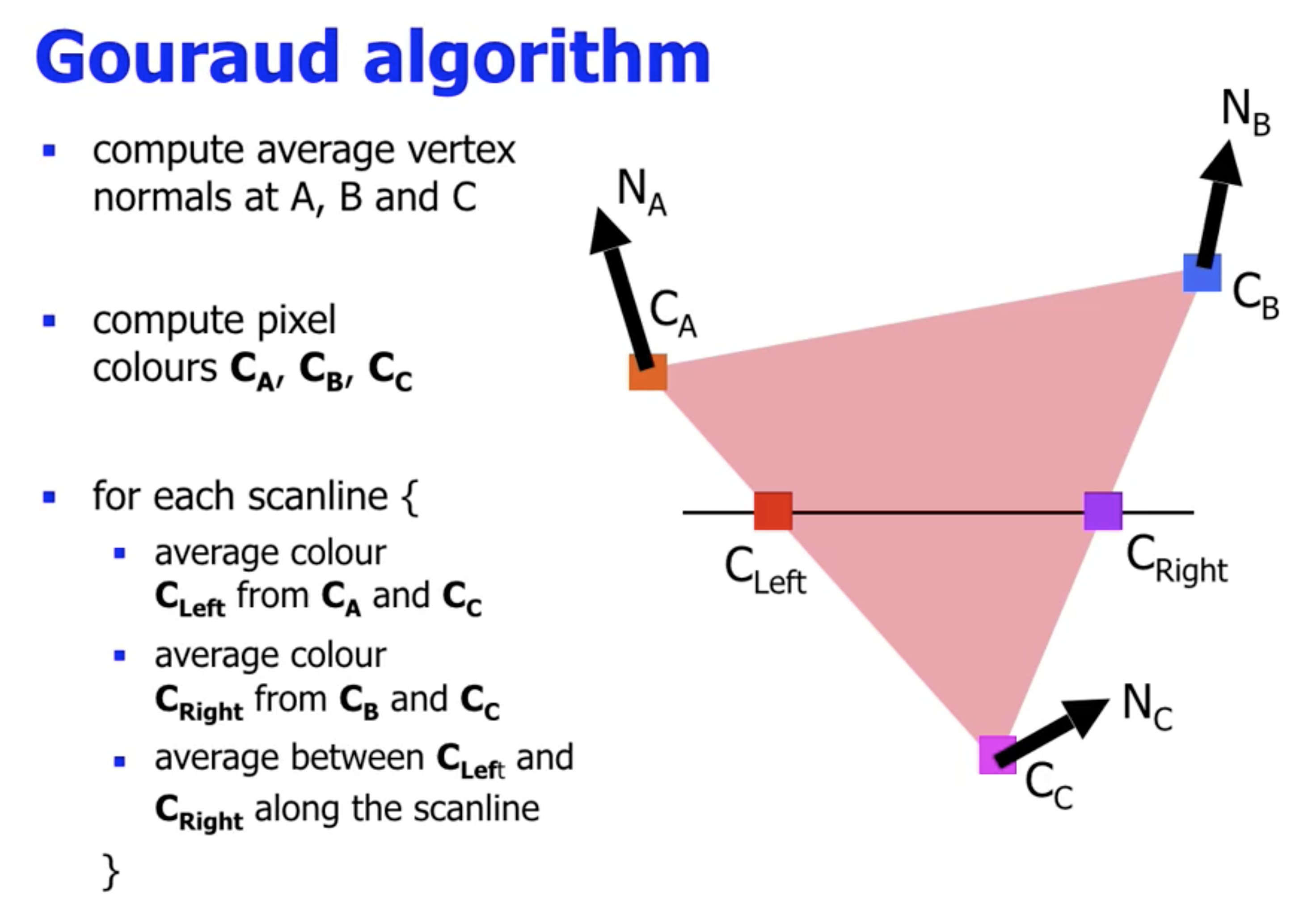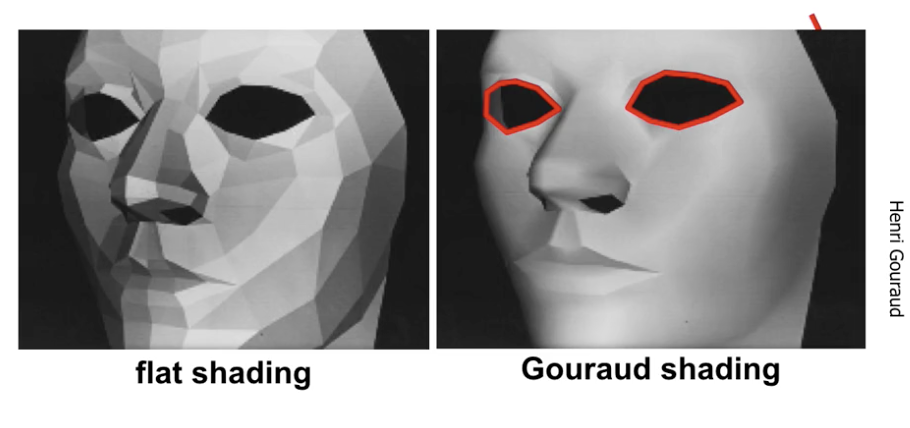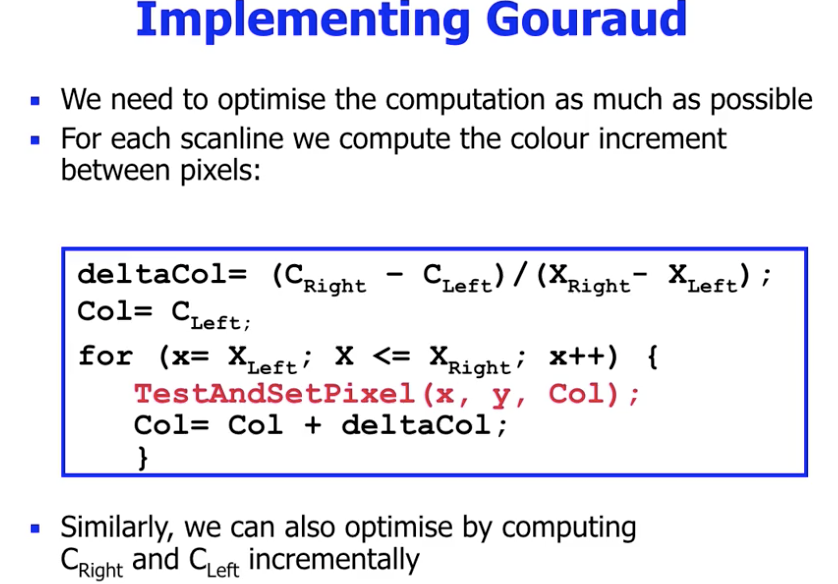1. 它在一些情况下可能 会将镜面高光平滑掉.
2. 它在一些情况下可能将 不该被平滑的边缘平滑掉.

### Phong Shading

Phong ShadingGouraud Shading 的不同之处在于: Gouraud Shading 直接基于顶点的颜色颜色进行平滑, 而 Phong Shading 先平滑法线向量 (Normal Vector), 然后再 基于法线向量分别计算每个像素的颜色.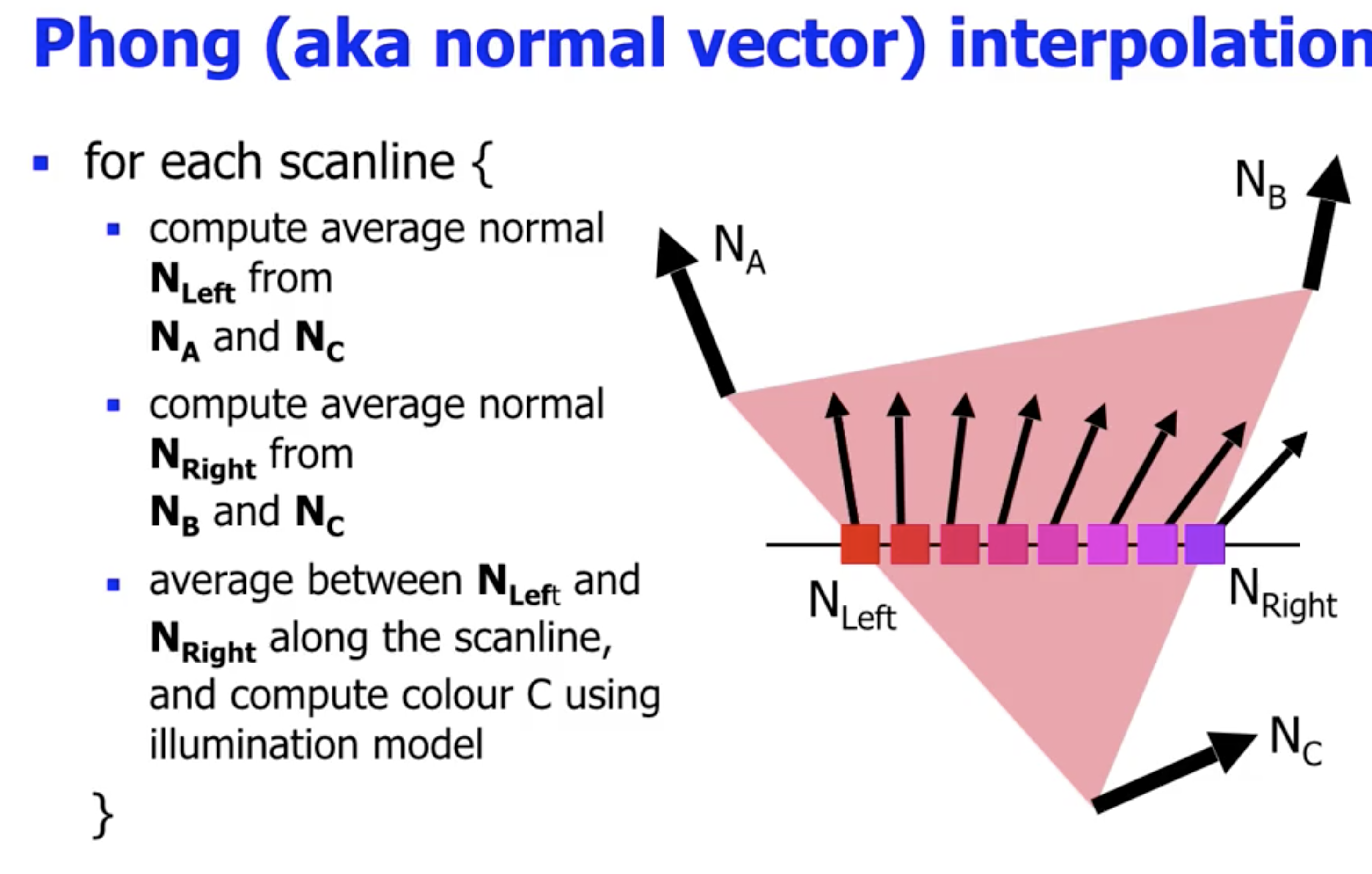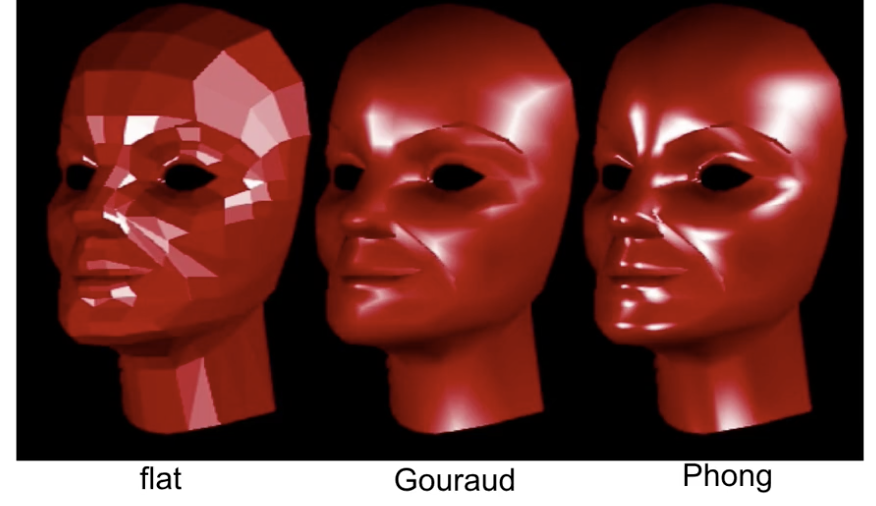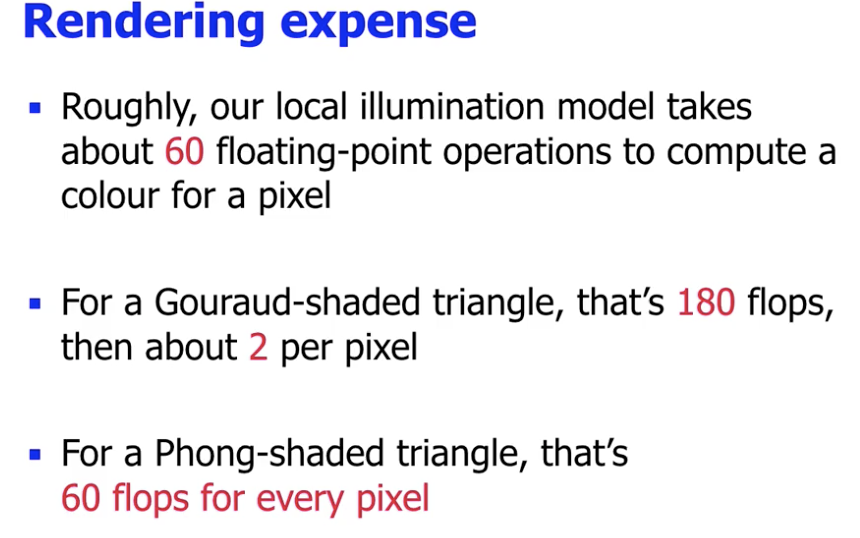## 贴图

### 纹理贴图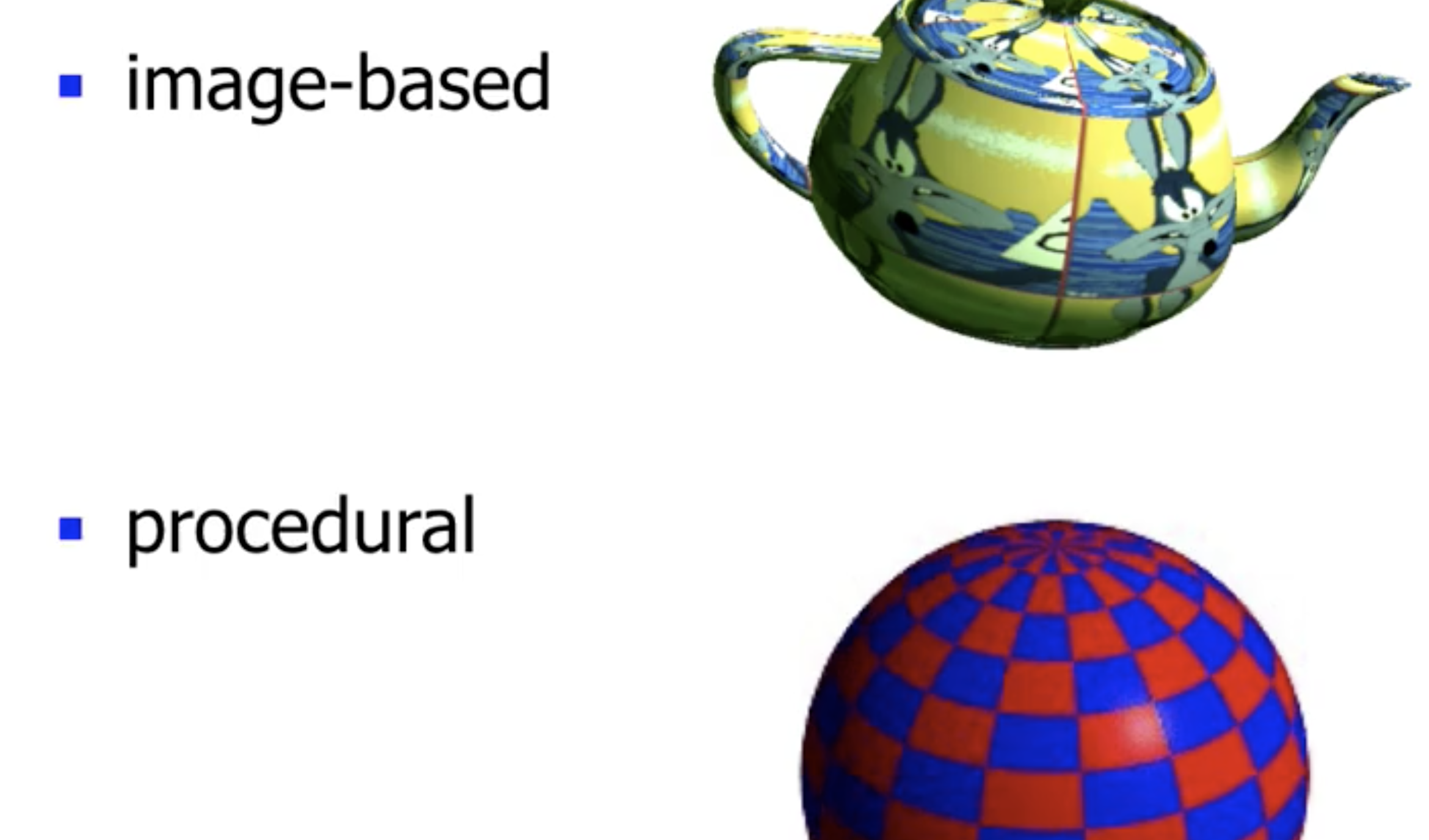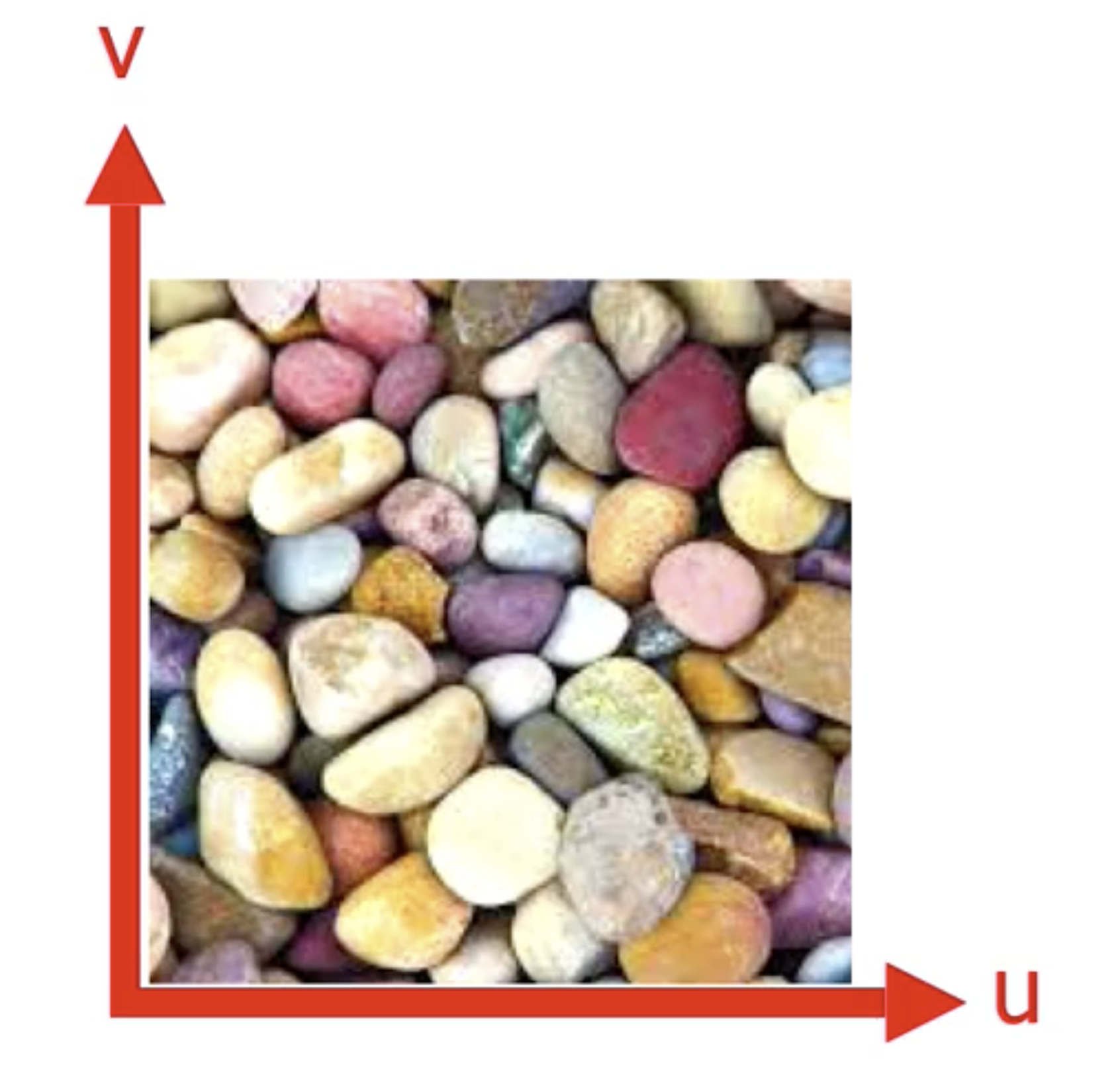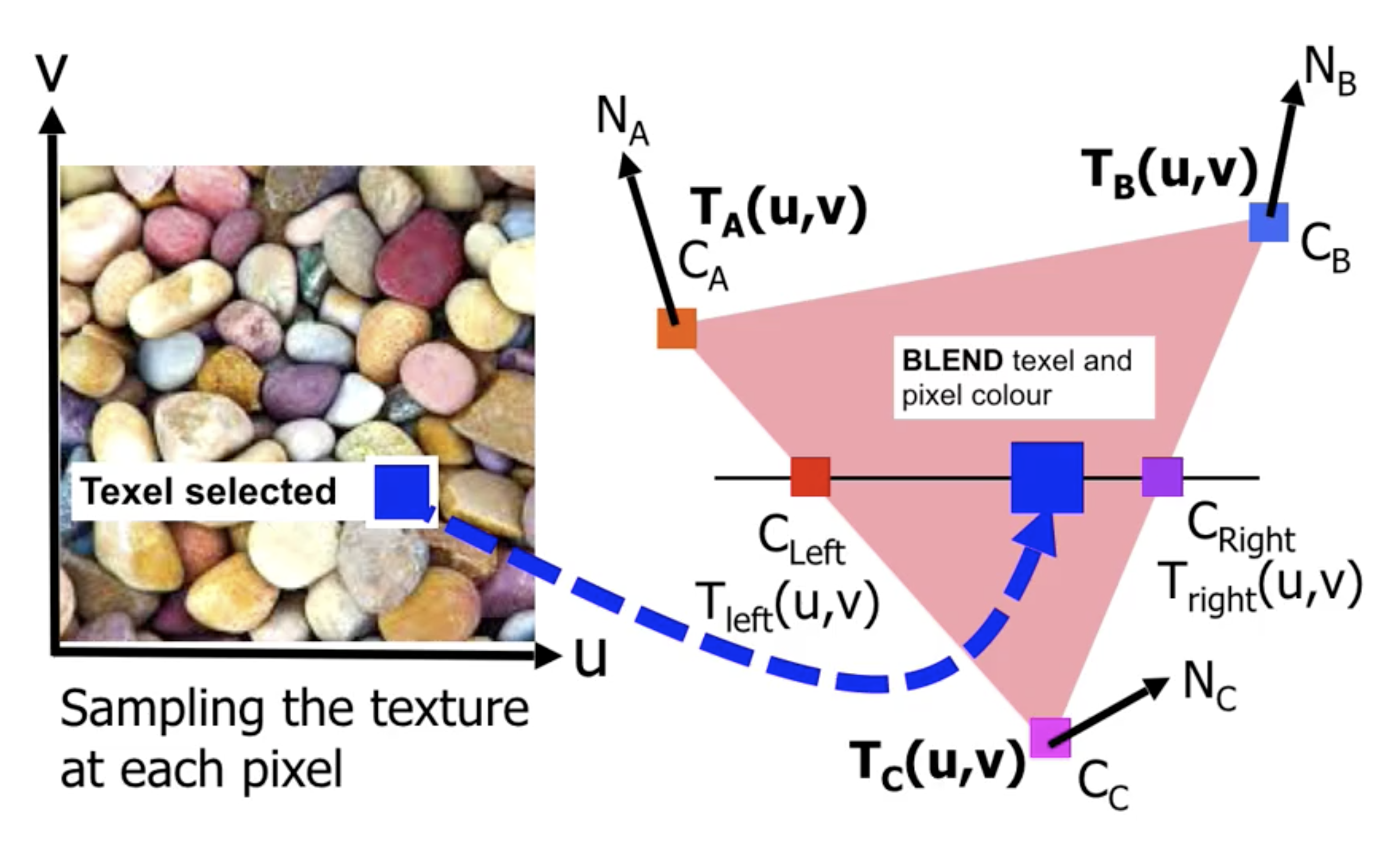#### 接缝问题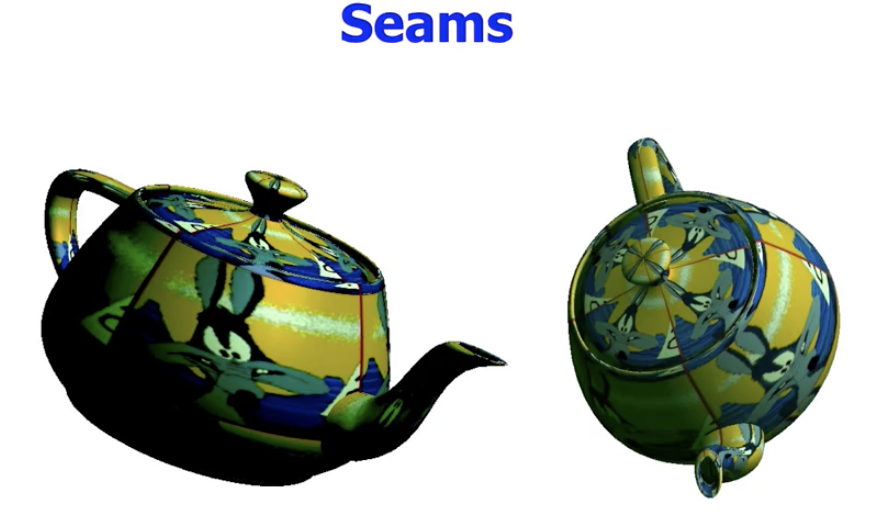#### 分辨率不一致问题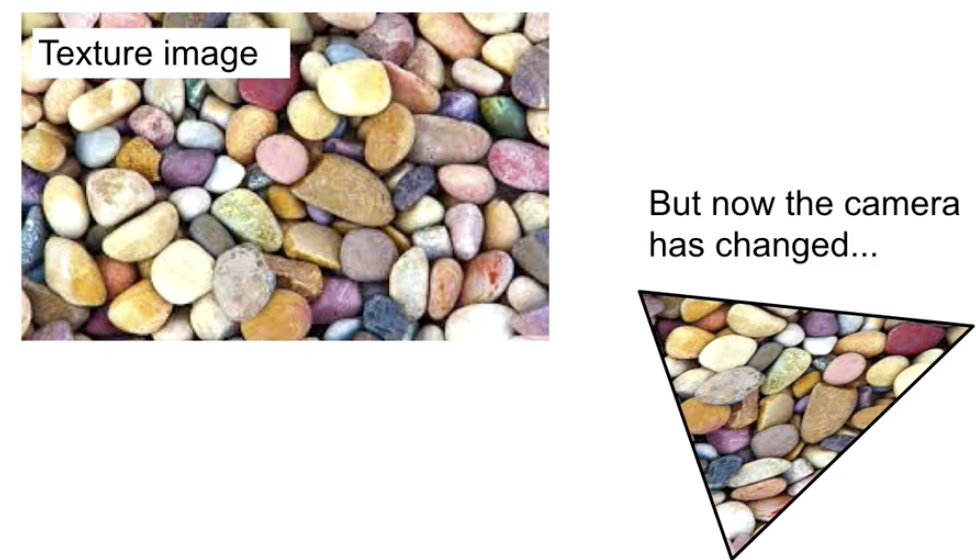##### 像素分辨率大于纹理分辨率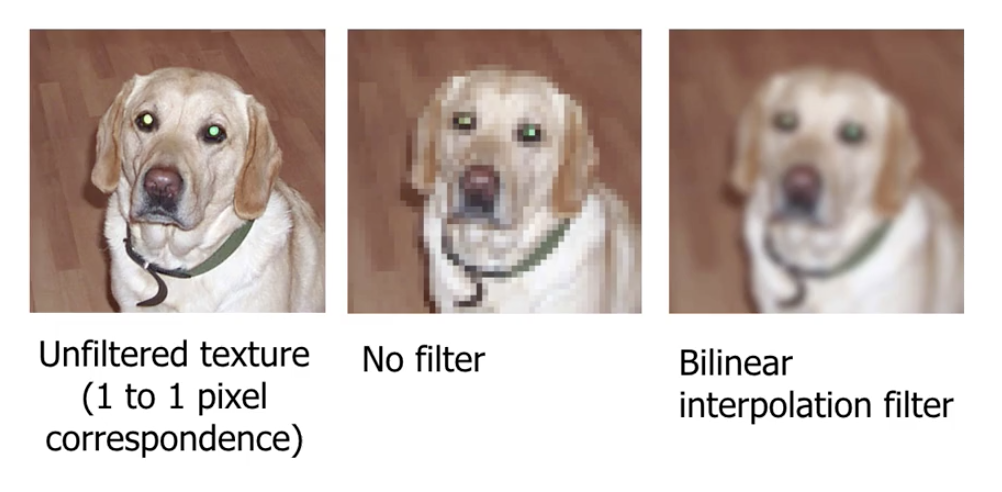###### 无过滤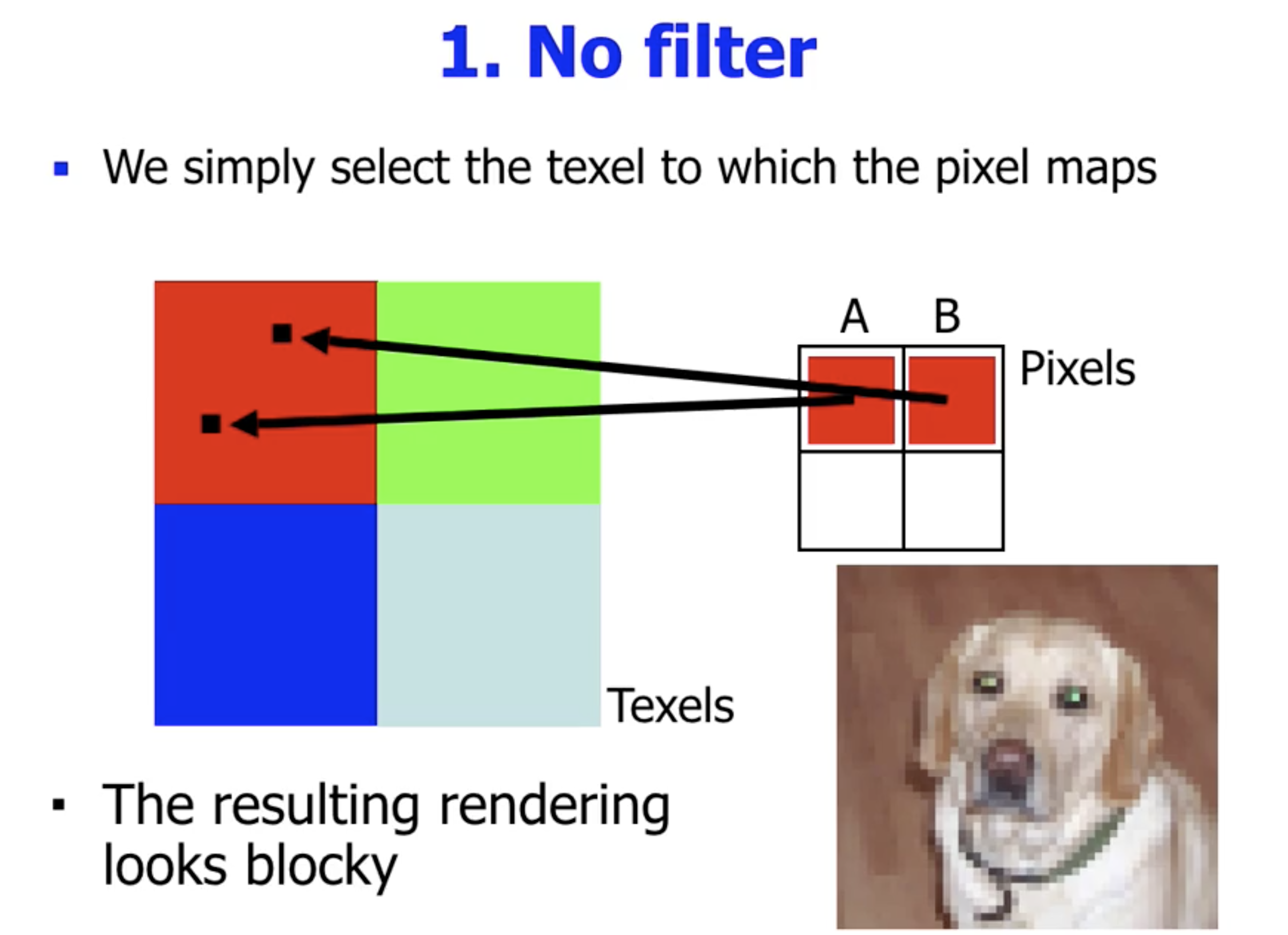###### 双线性插值过滤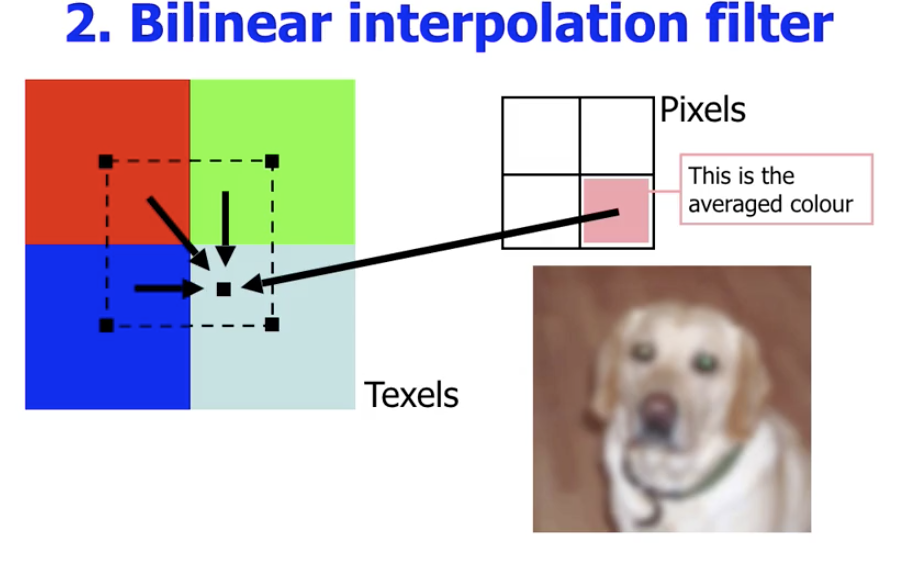##### 像素分辨率小于纹理分辨率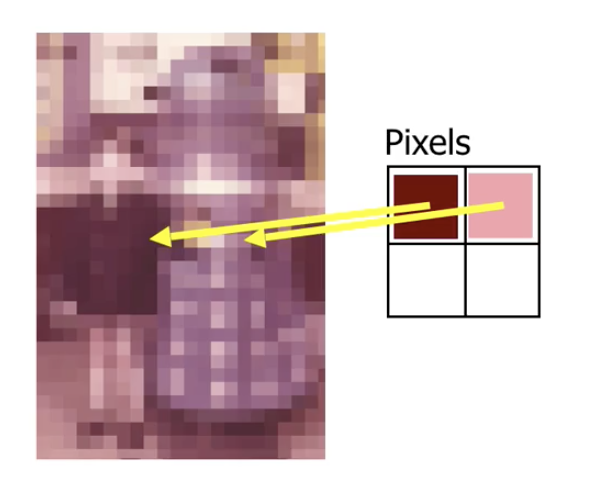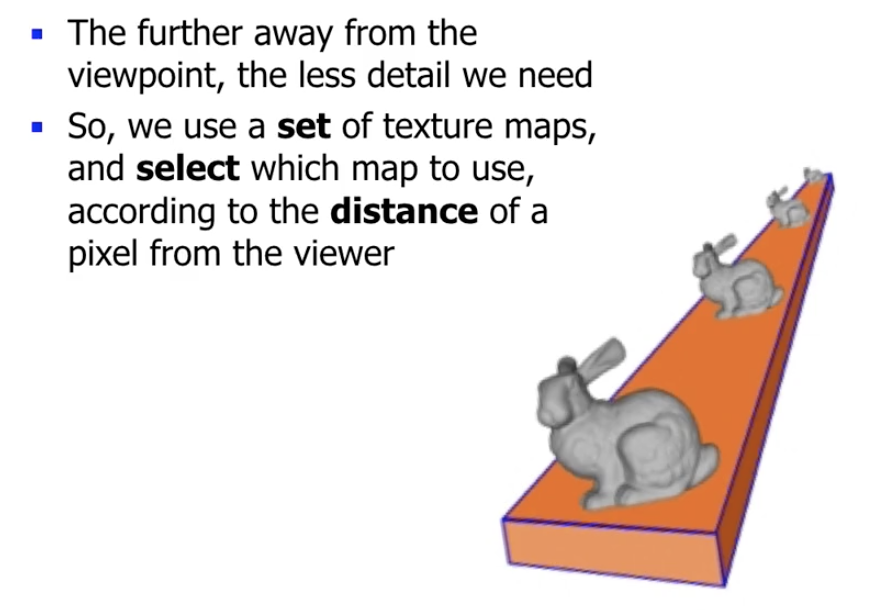(The origin of the term mipmap is an initialism of the Latin phrase multum in parvo (“much in a small space”)”)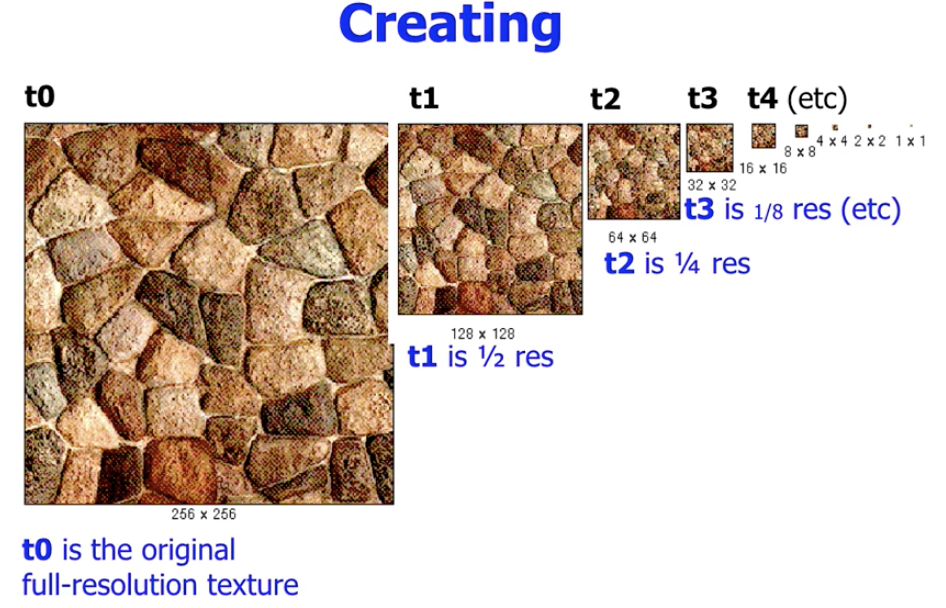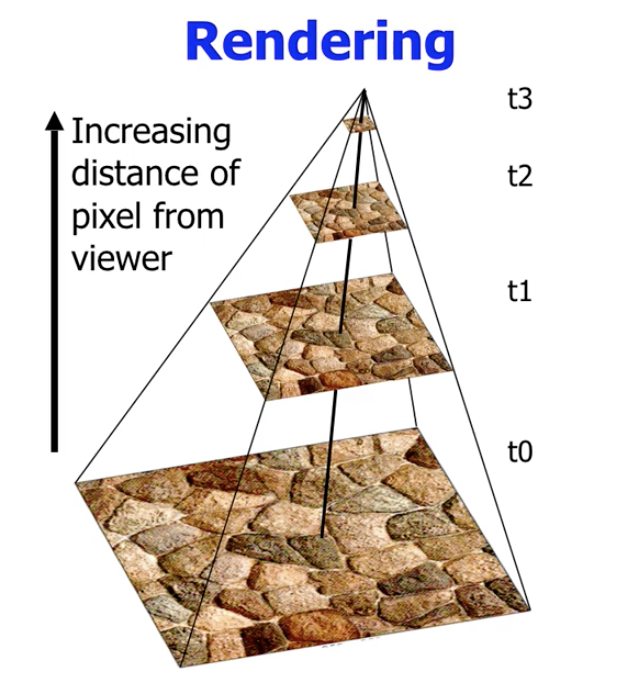#### 纹理贴图的其他用处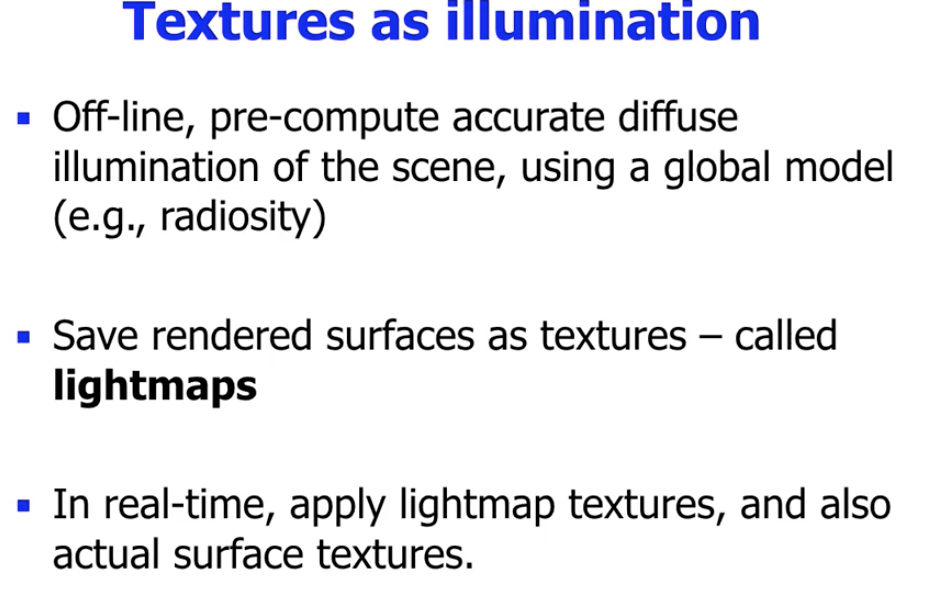### 凹凸贴图